# RS Aggarwal Solutions for Class 10 Maths Chapter 1 Real Numbers

## RS Aggarwal Solutions for Class 10 Chapter 1 Real Numbers

RS Aggarwal Solutions for Class 10 Chapter 1 Real Numbers are prepared by BYJU’S Subject expert based on the latest CBSE syllabus. We provide solutions for all the questions enlisted under the chapter. Practice real numbers RS Aggarwal questions and get ready for any exam. All solutions are explained in a step-by-step manner. Download RS Aggarwal Maths Class 10 Solutions and start practicing offline.

## Download PDF of RS Aggarwal Solutions for Class 10 Chapter 1 Real Numbers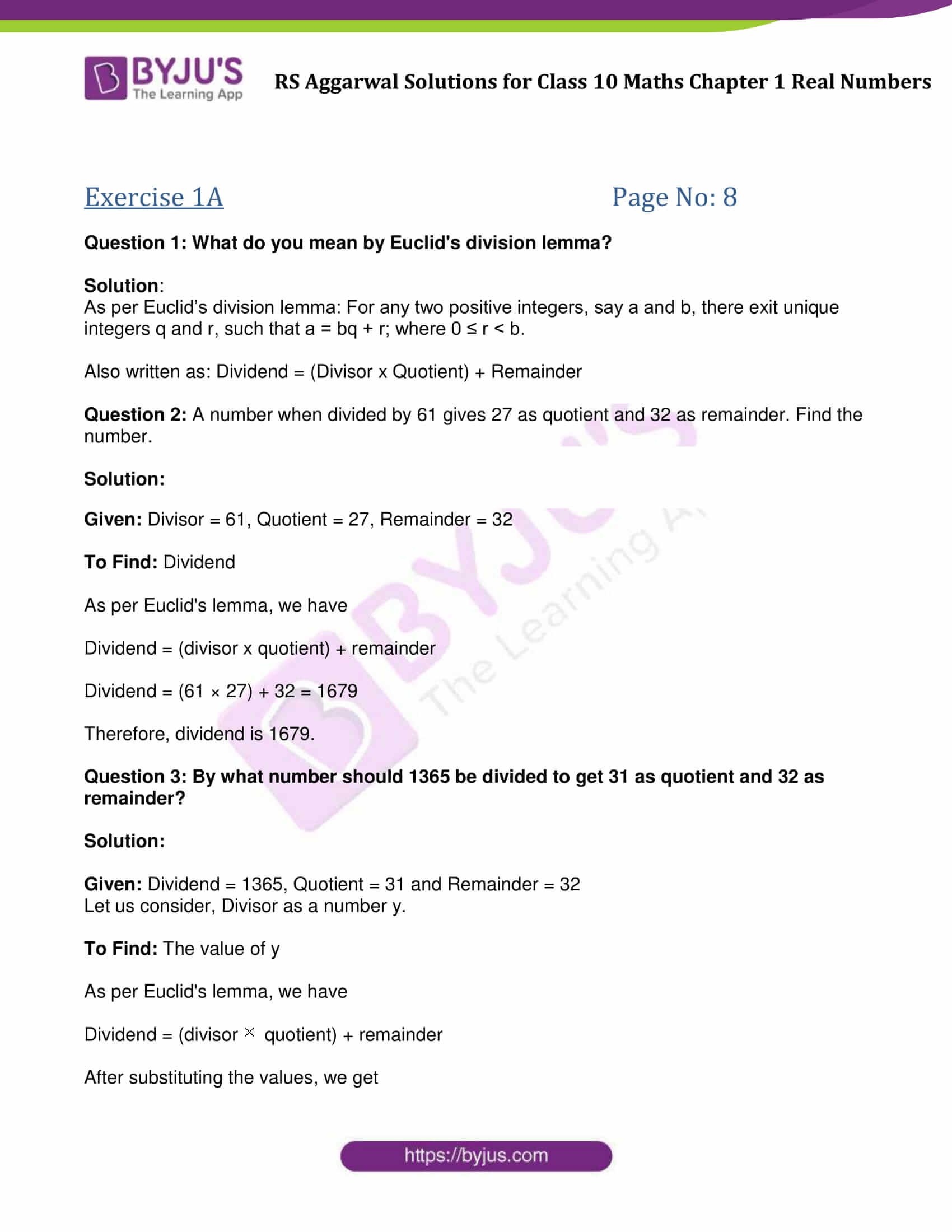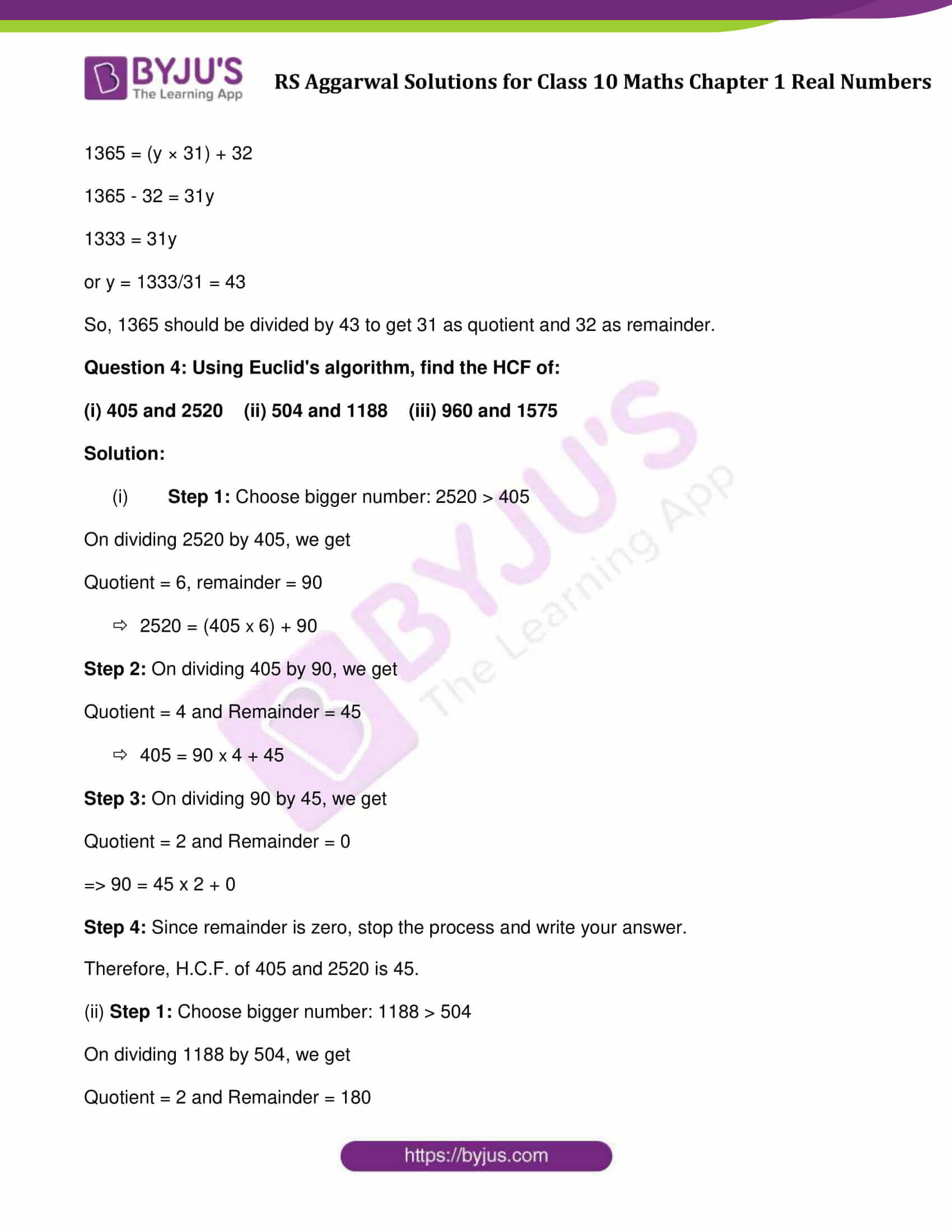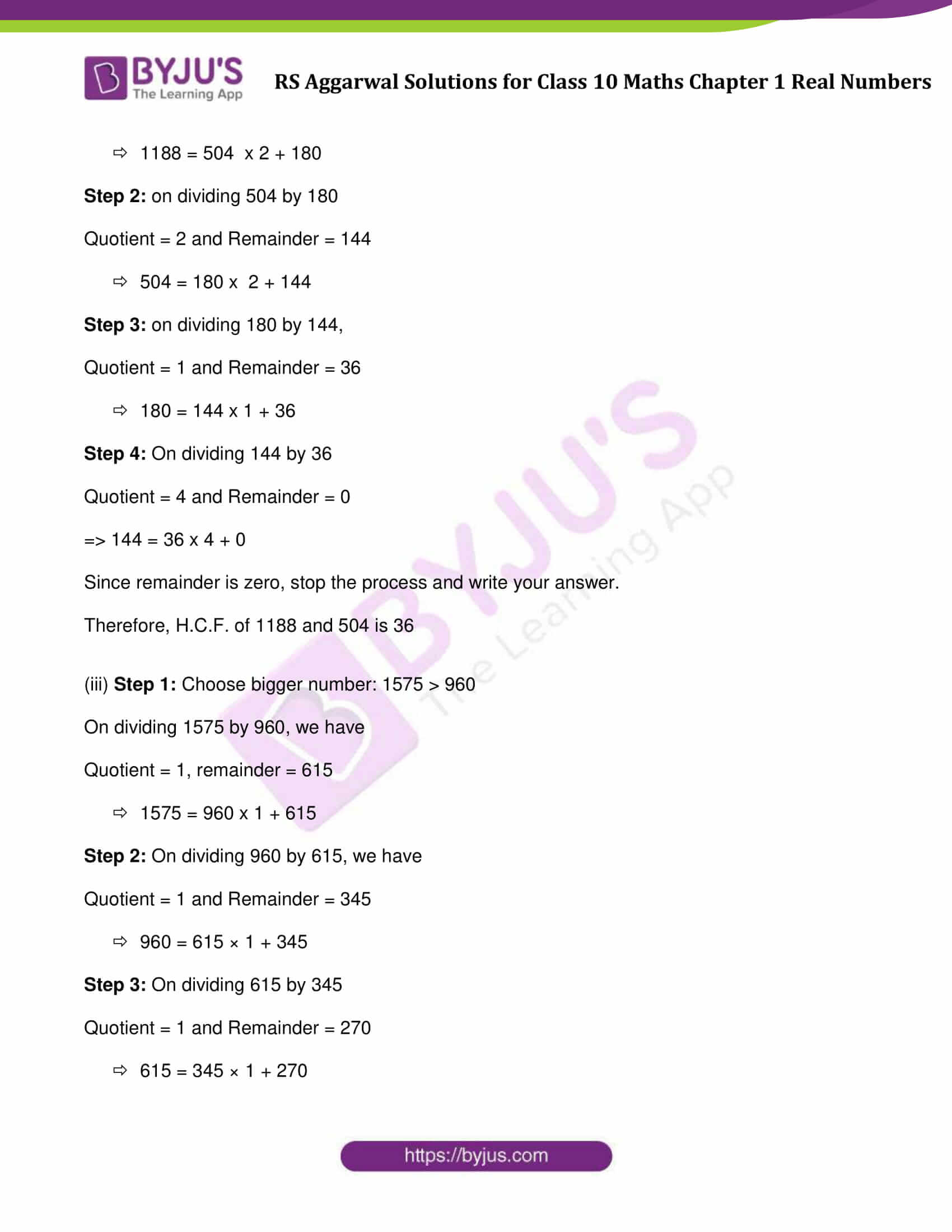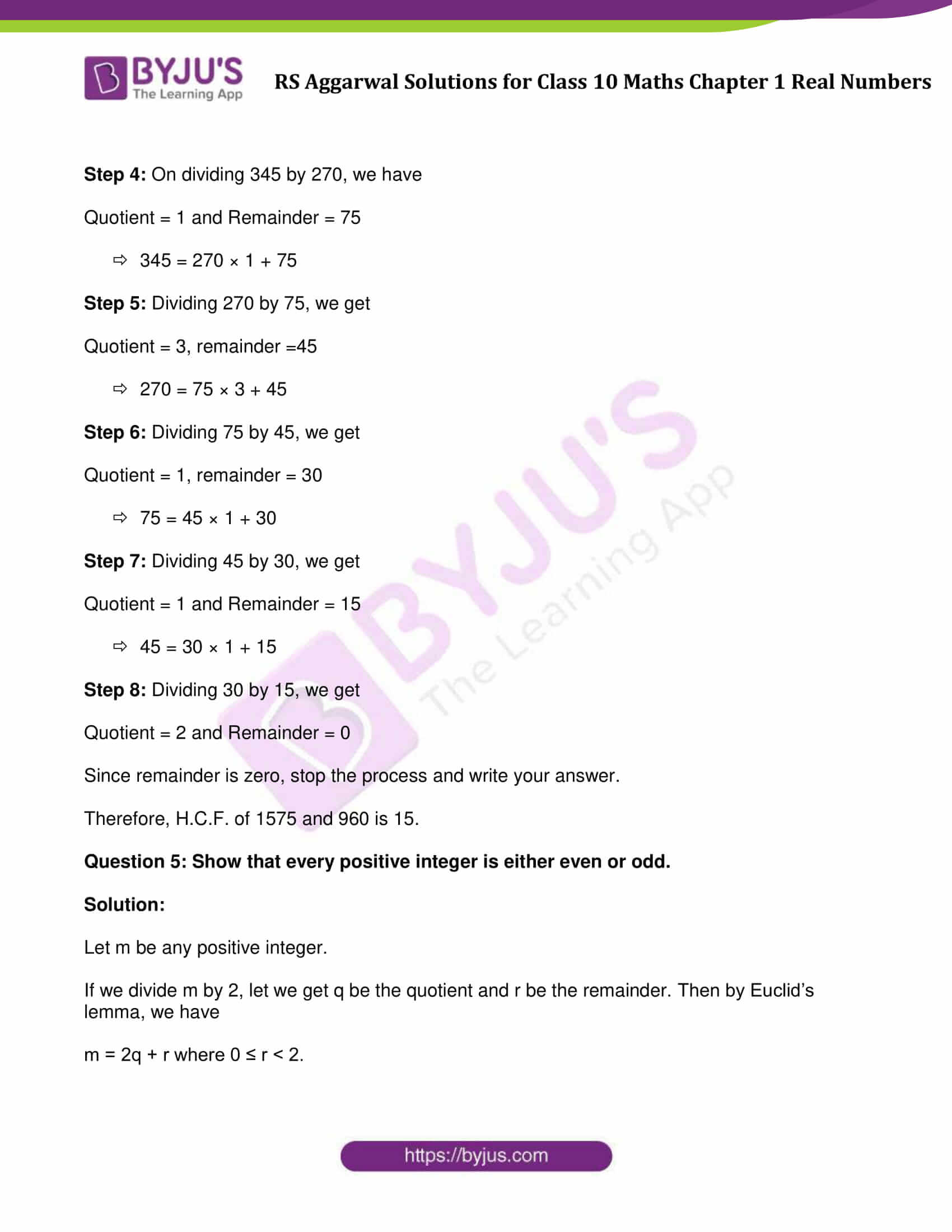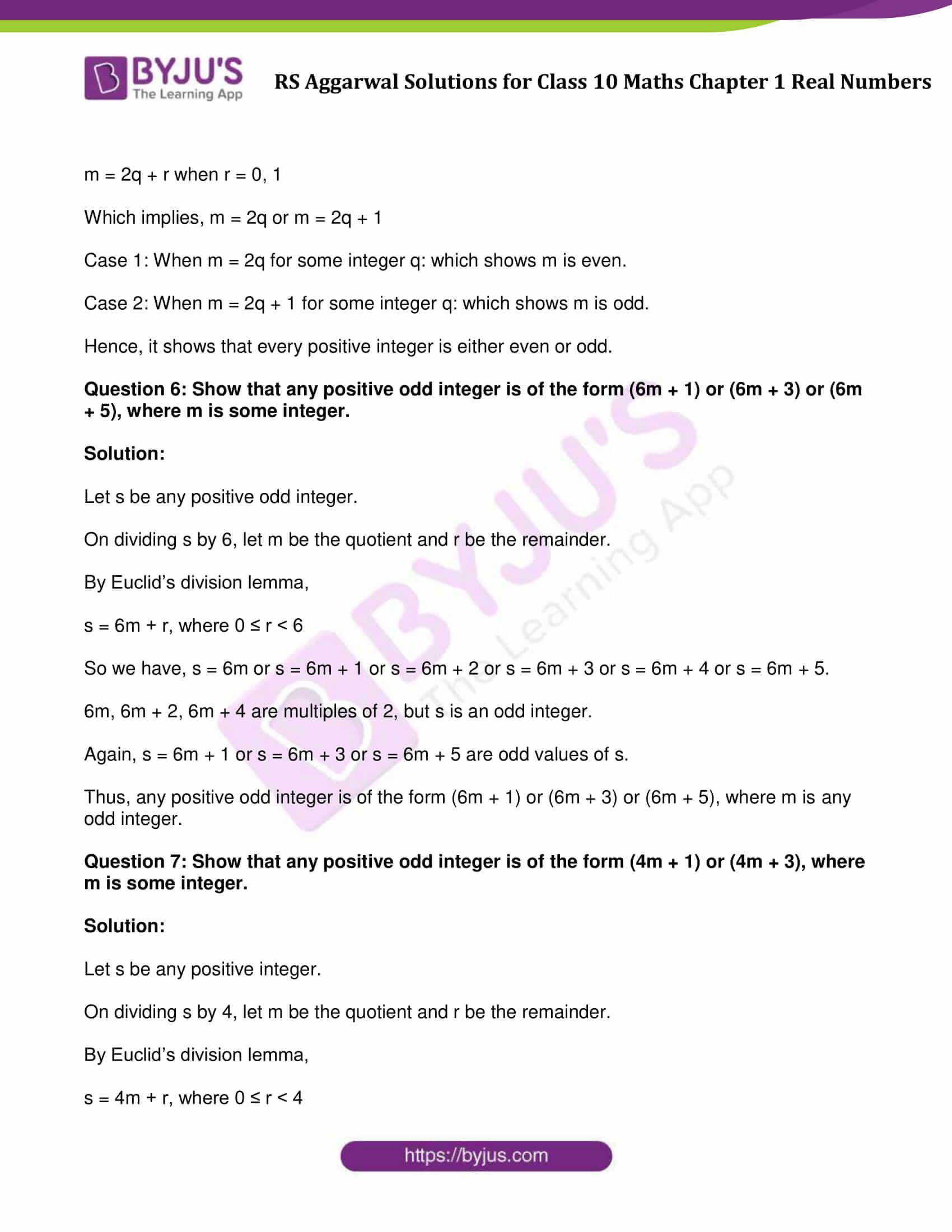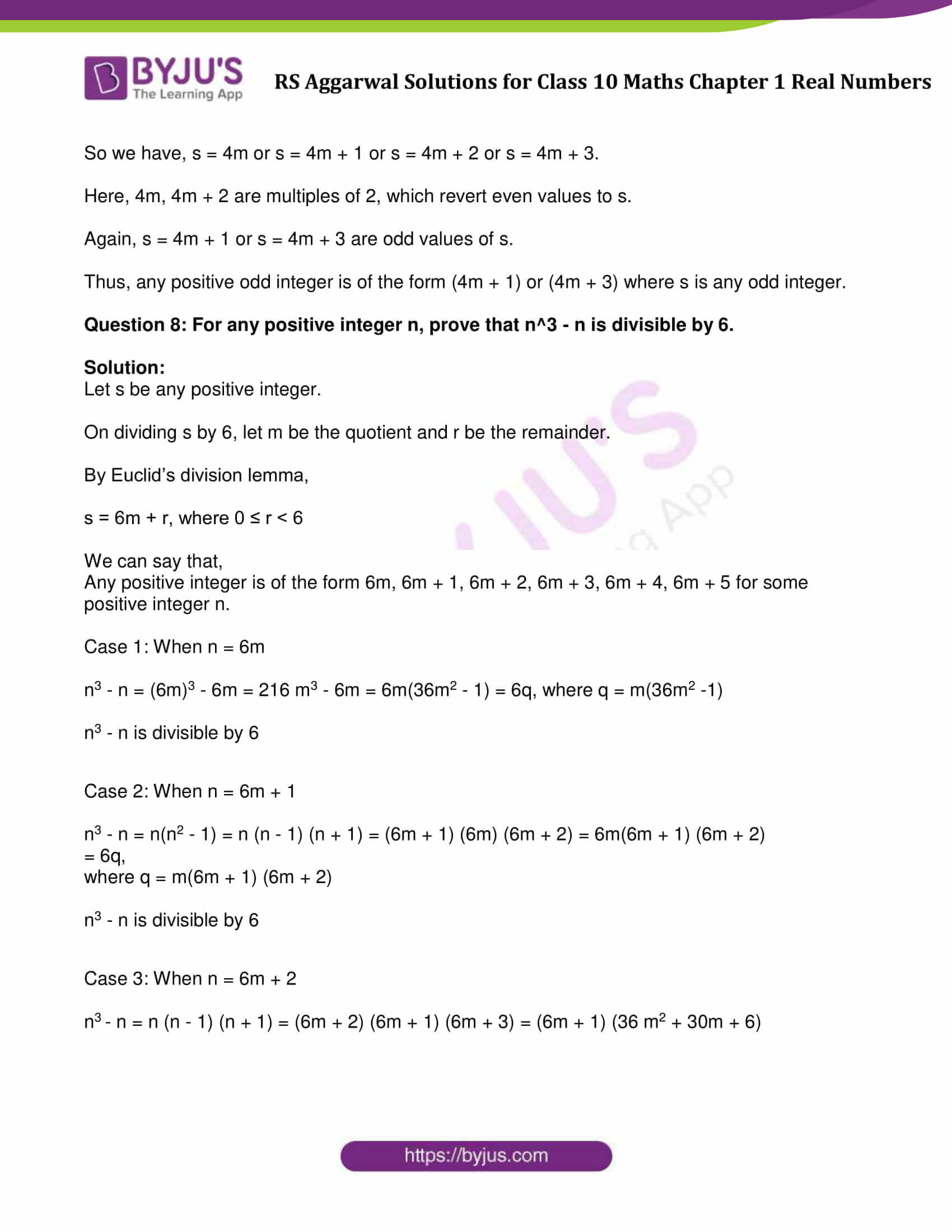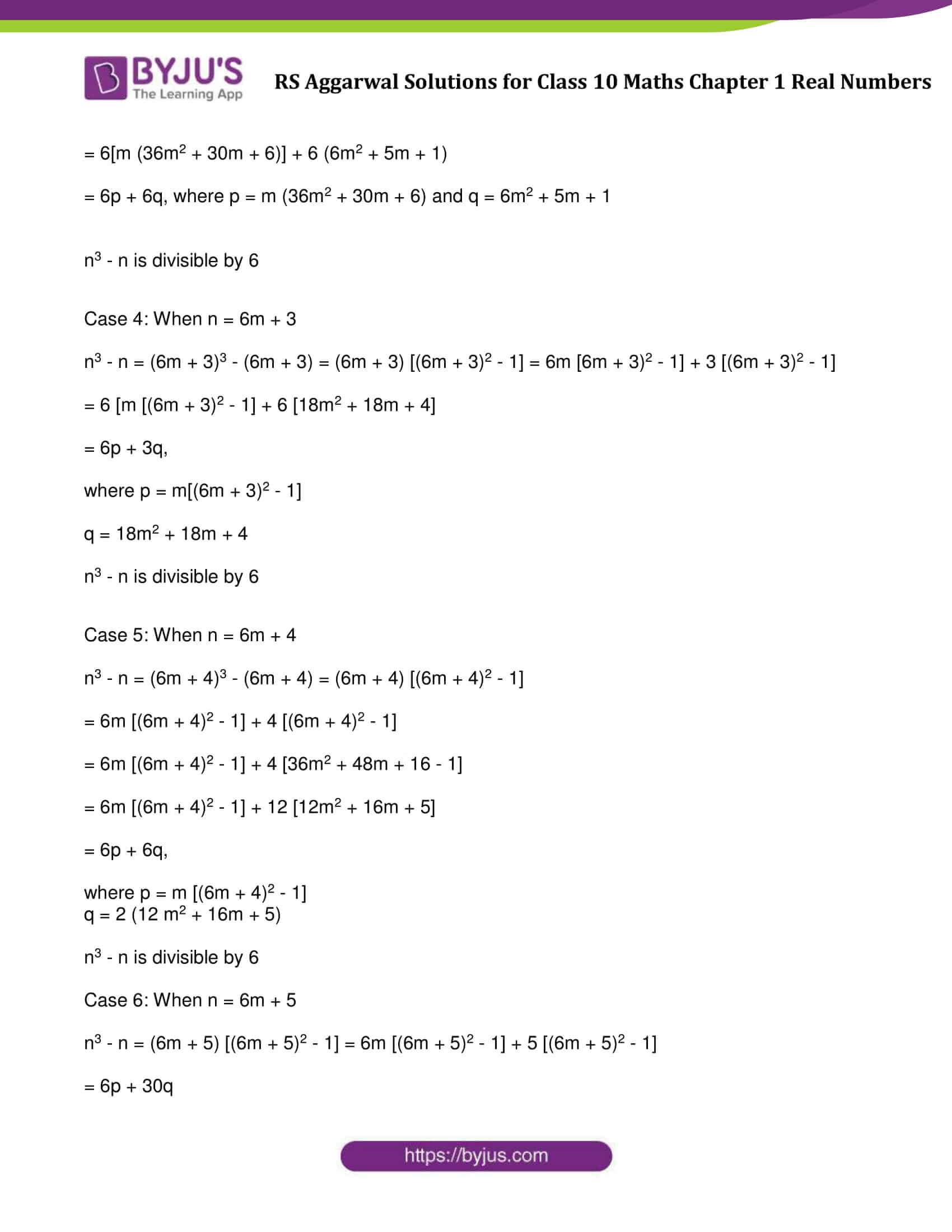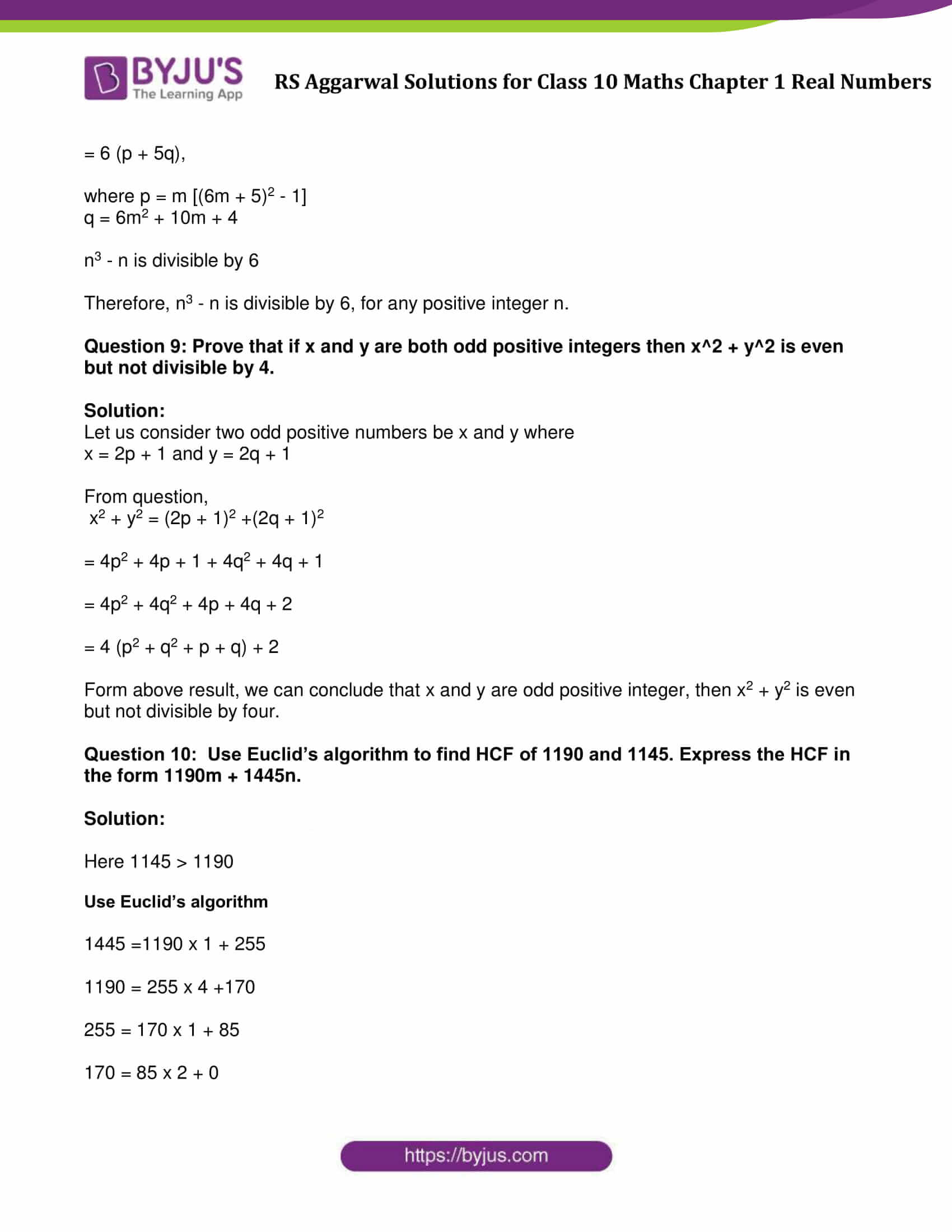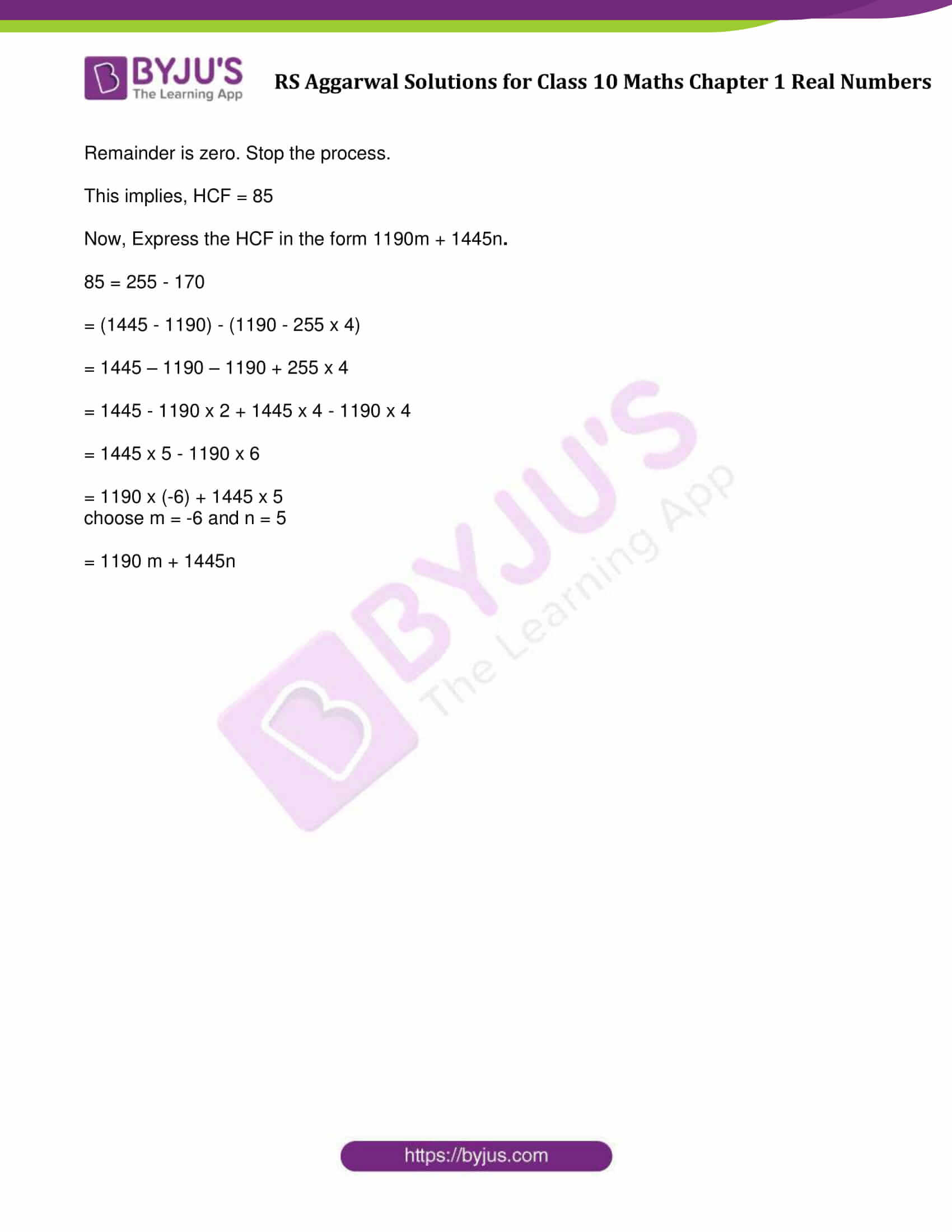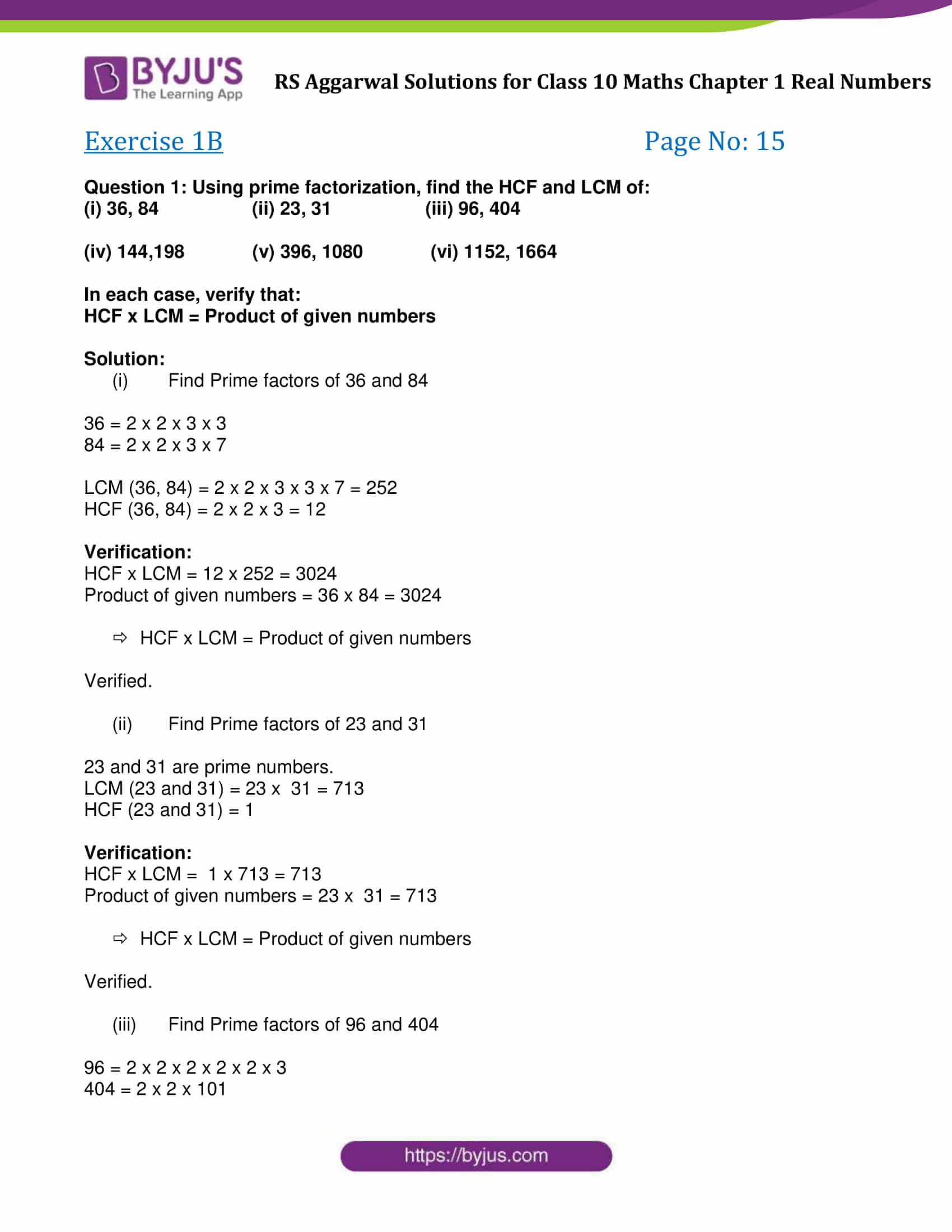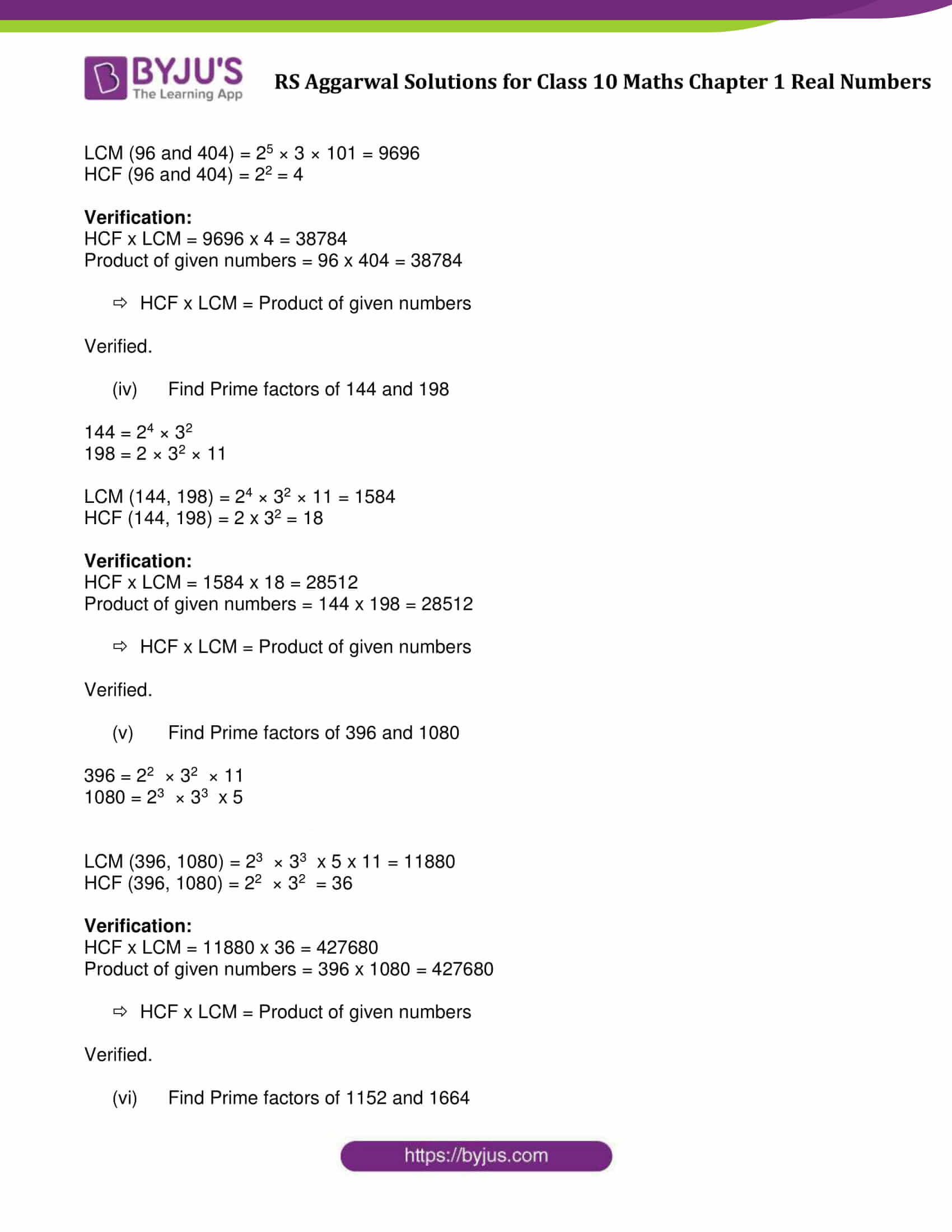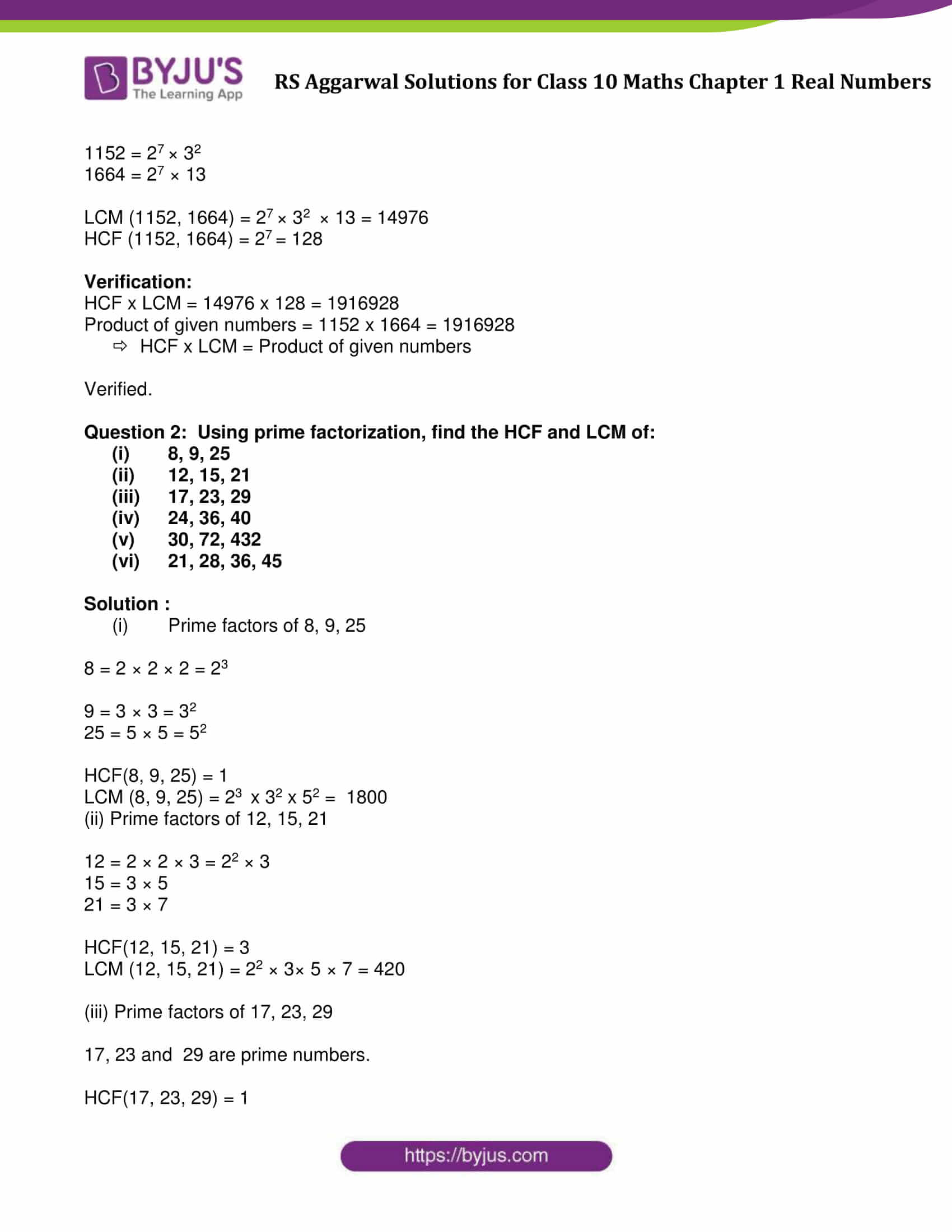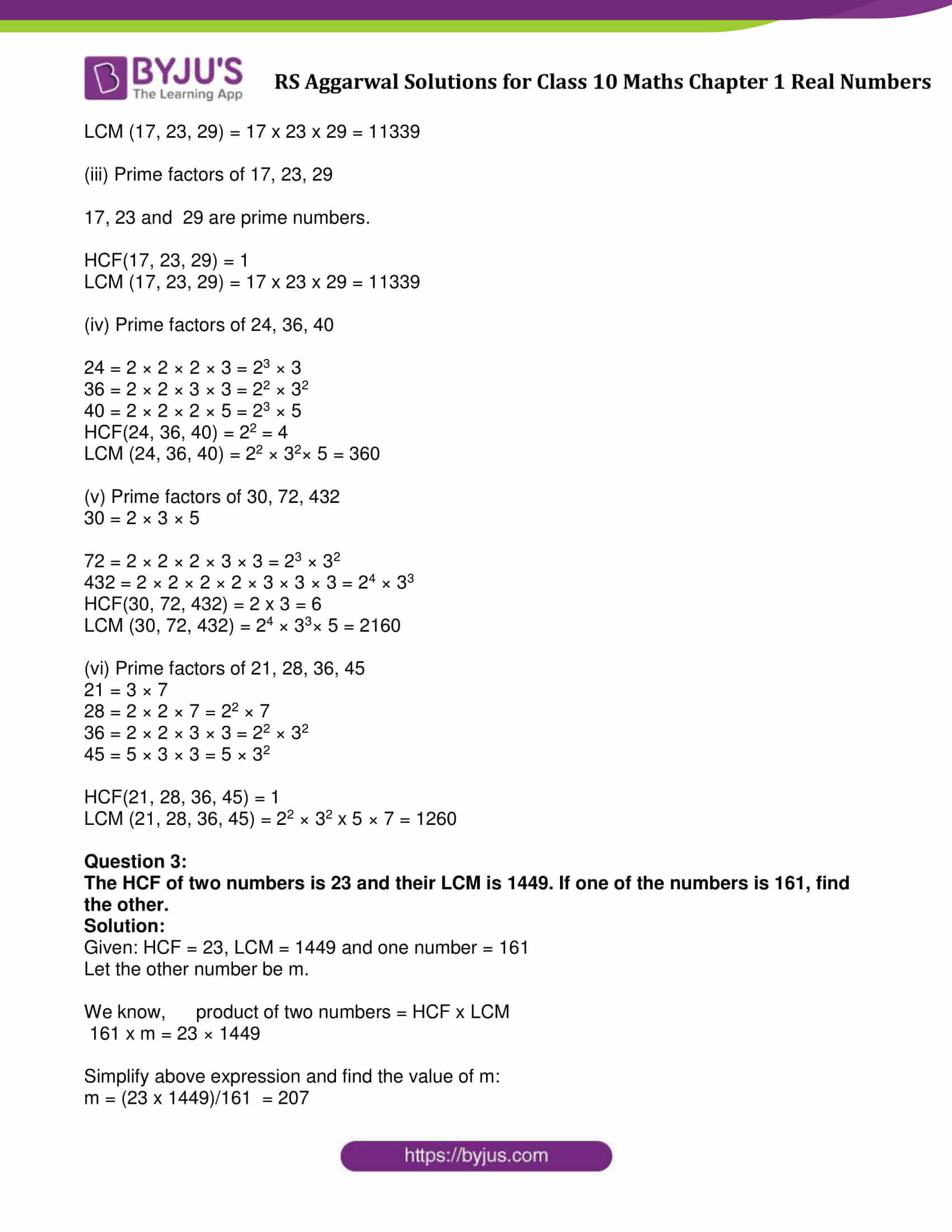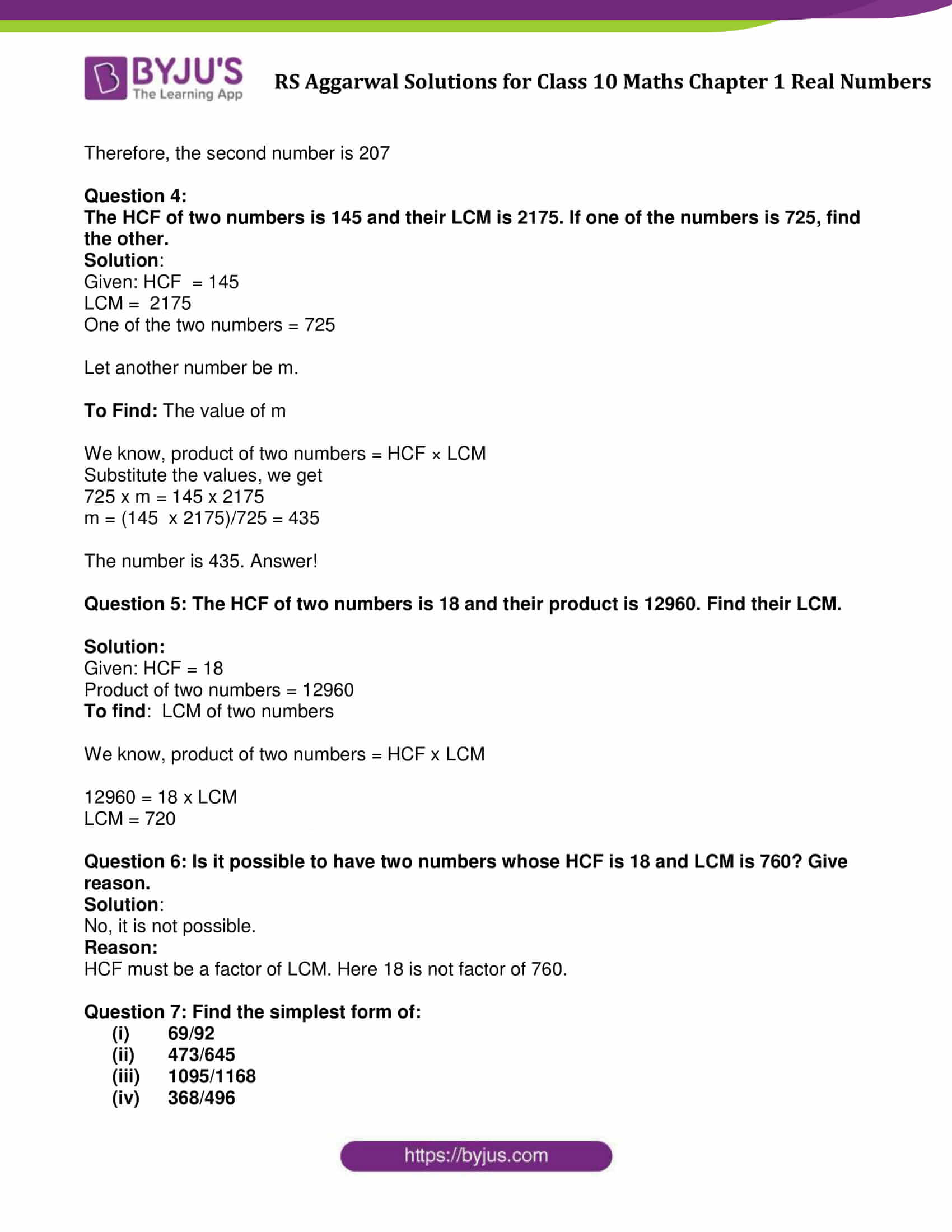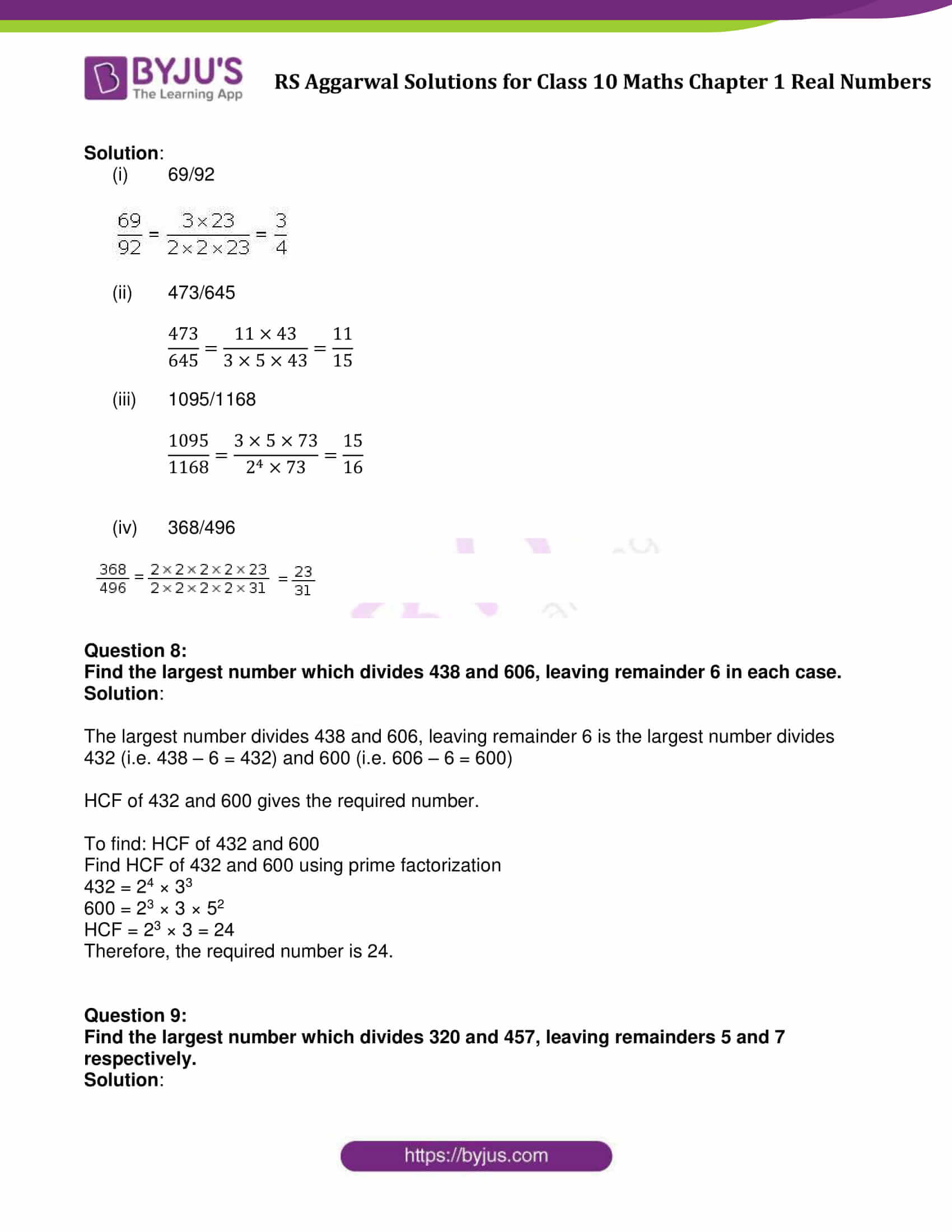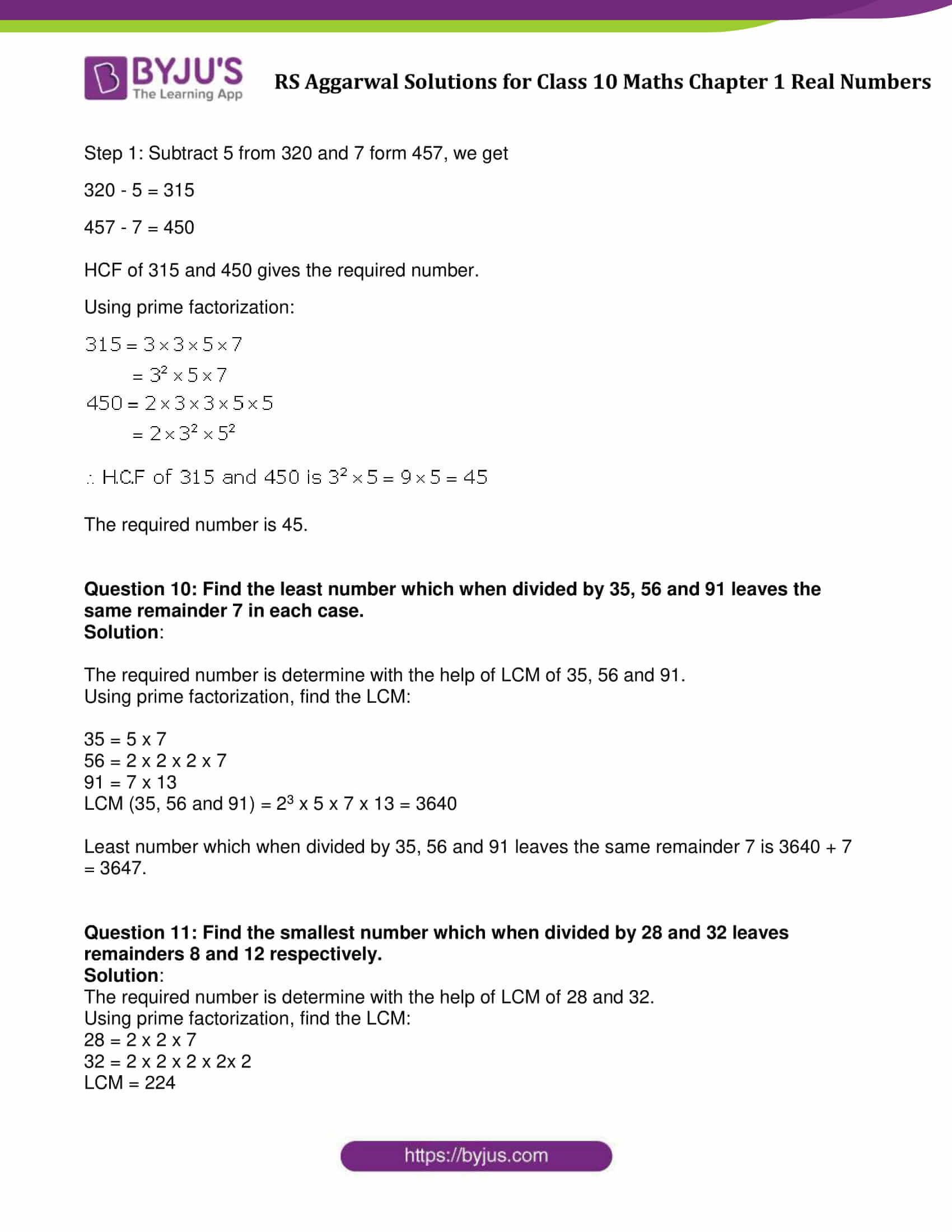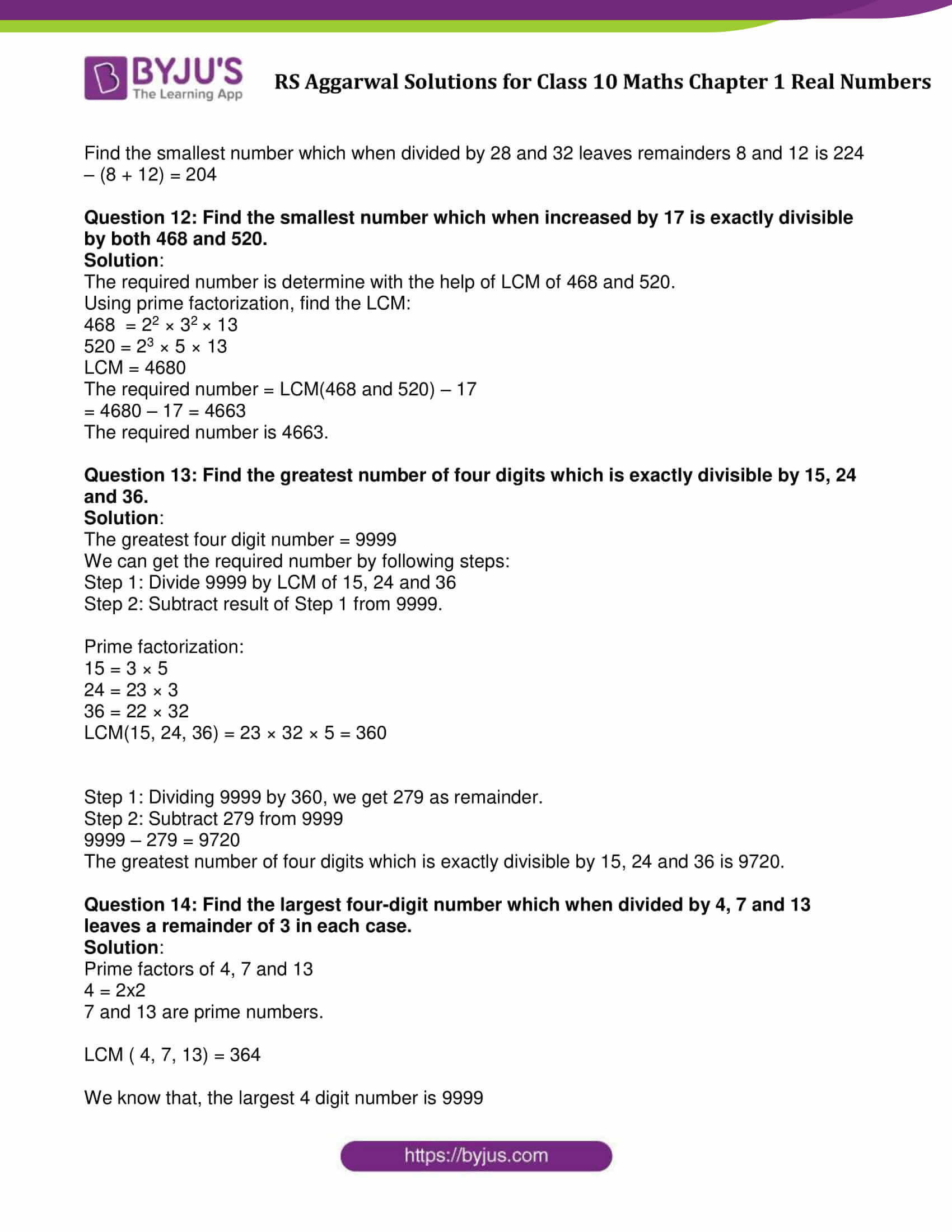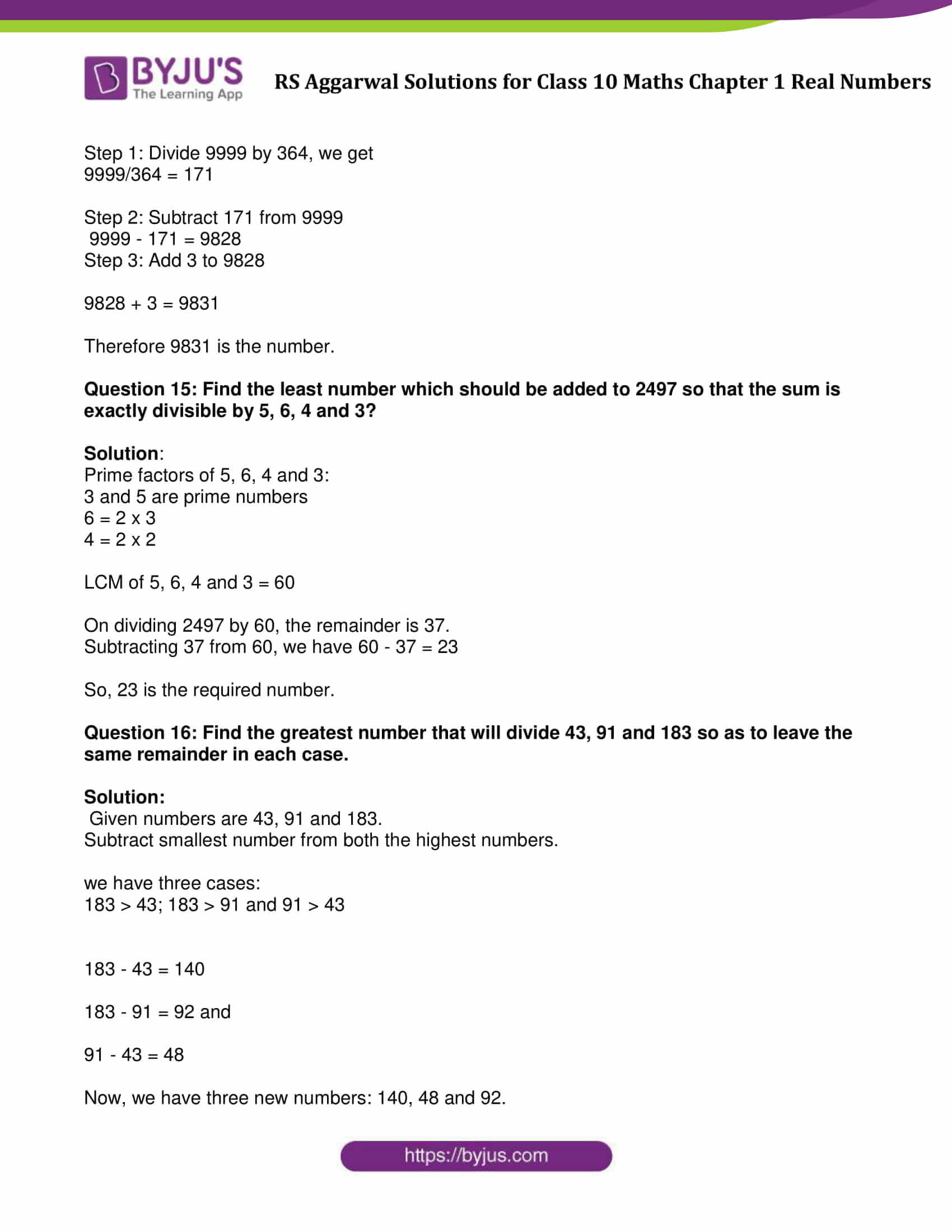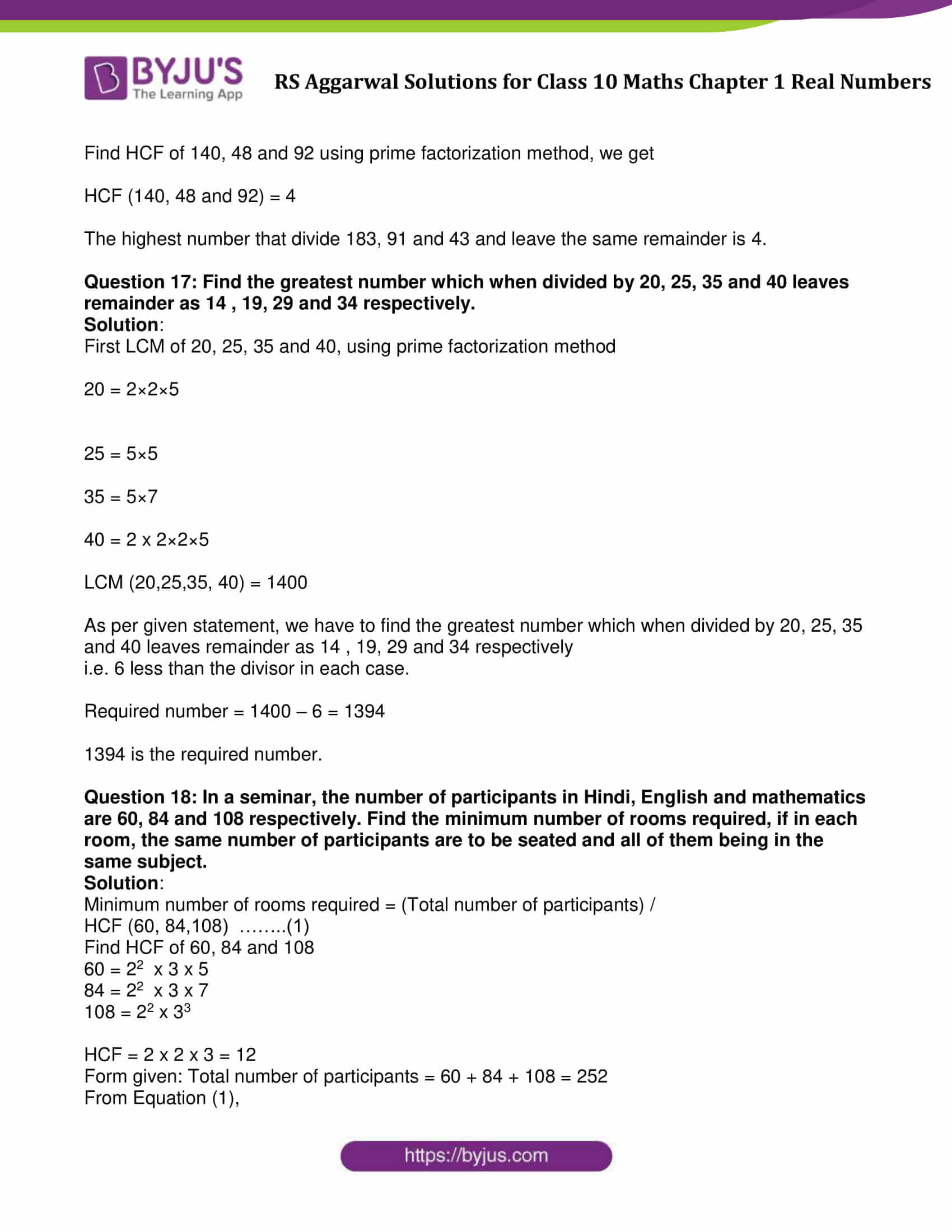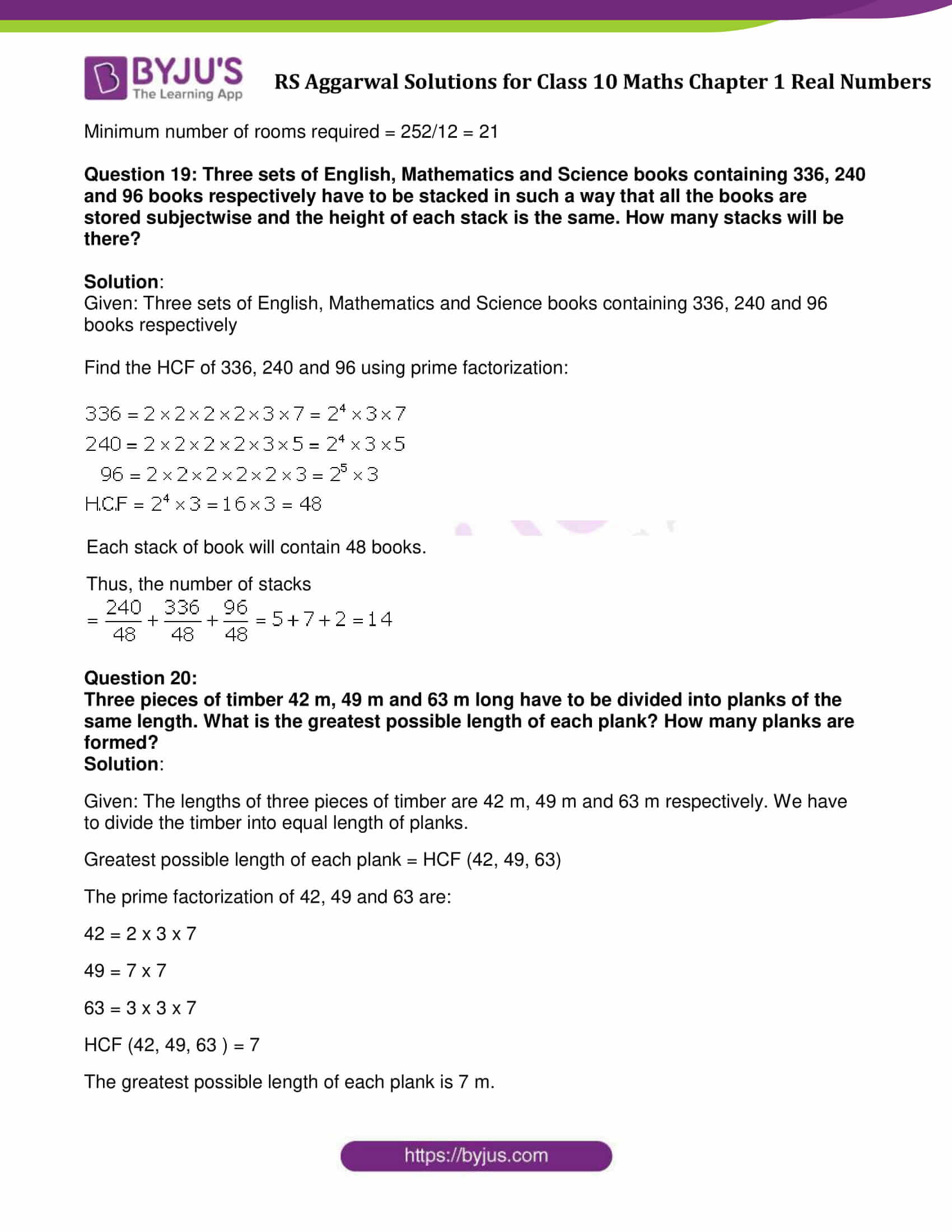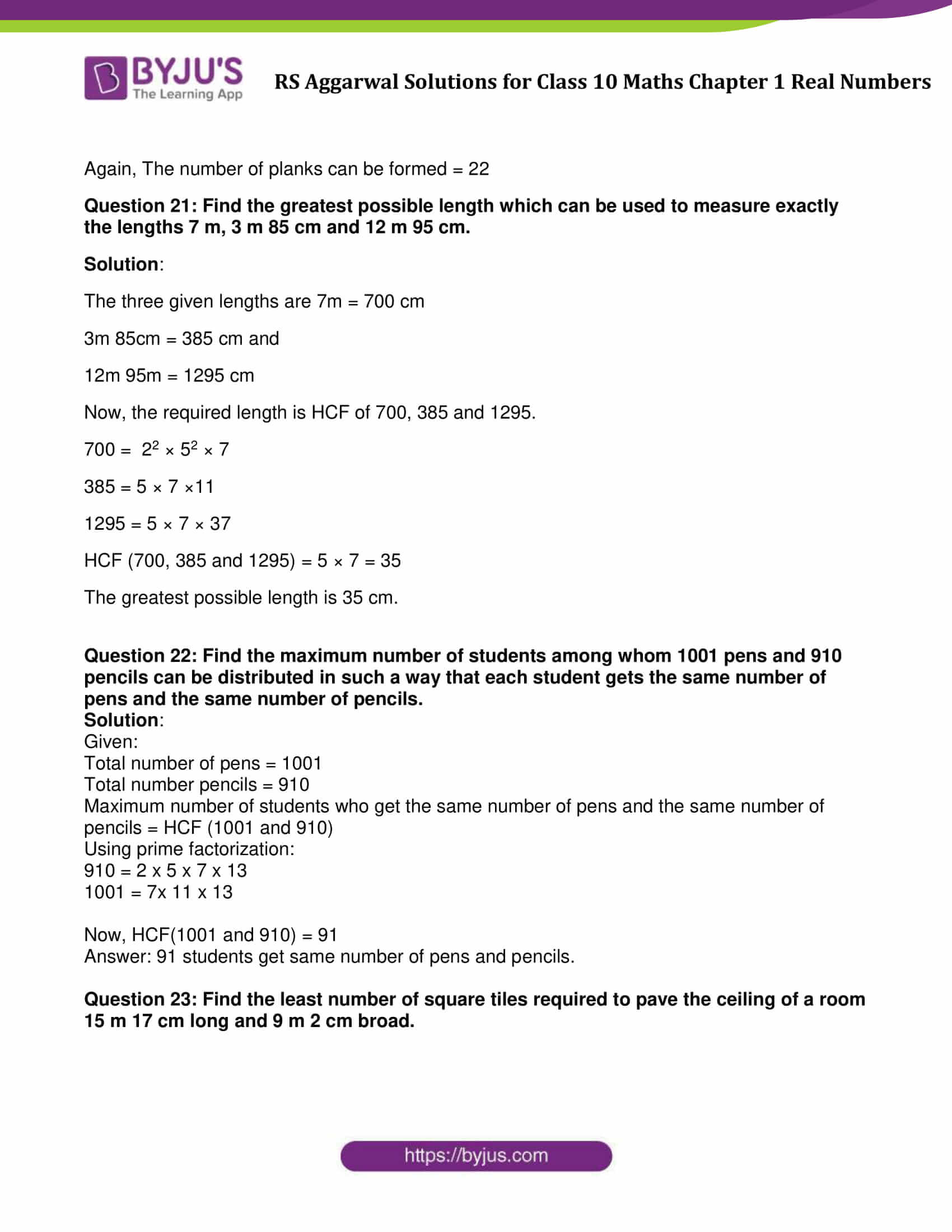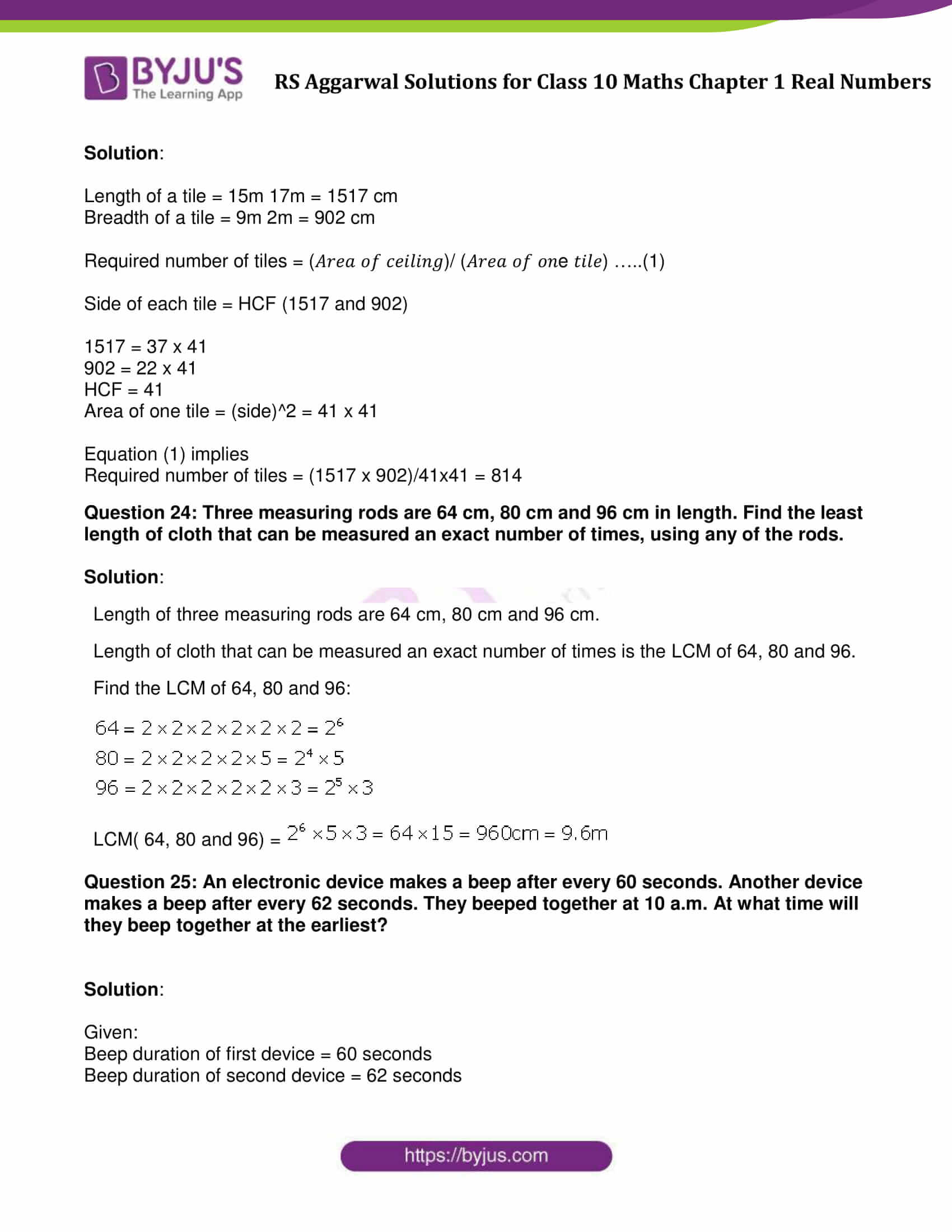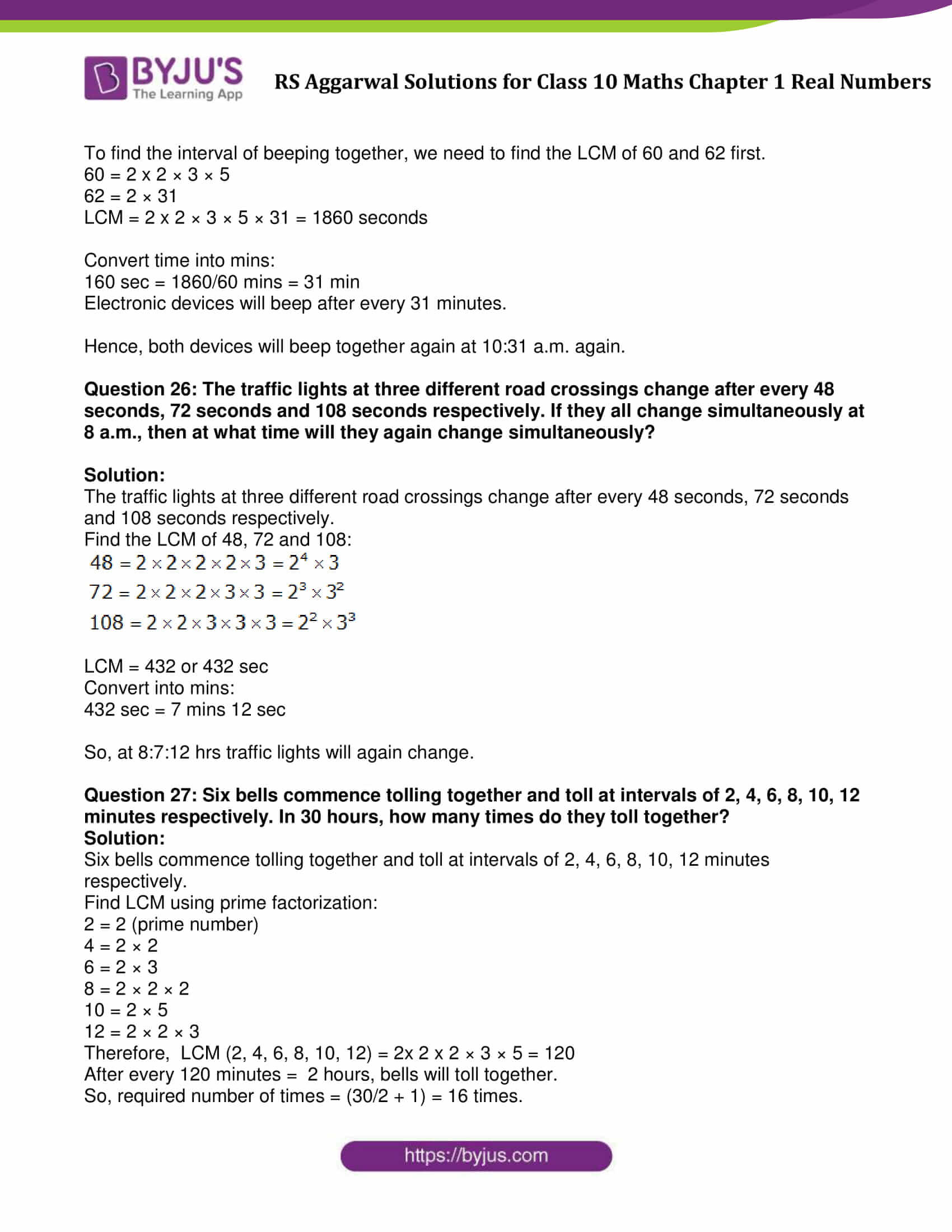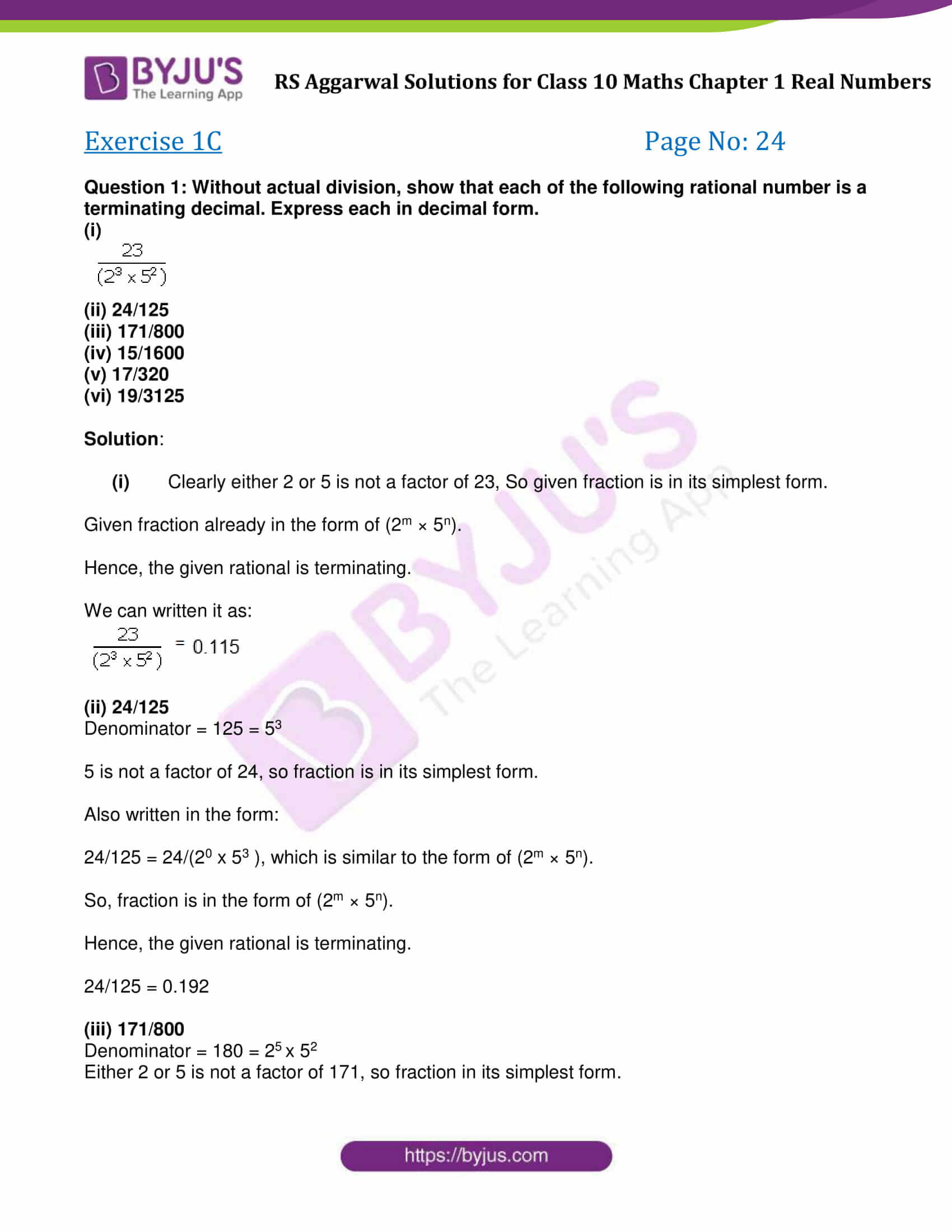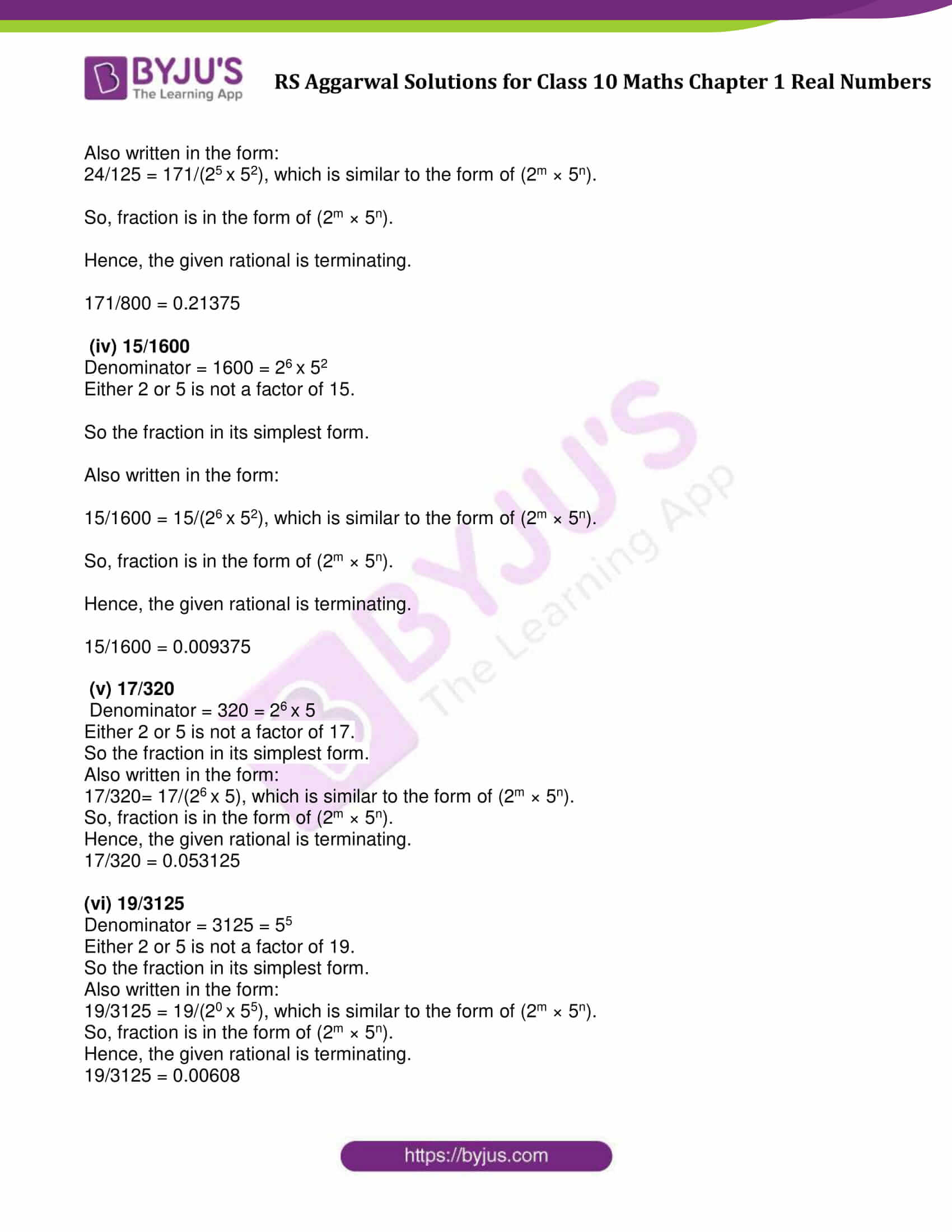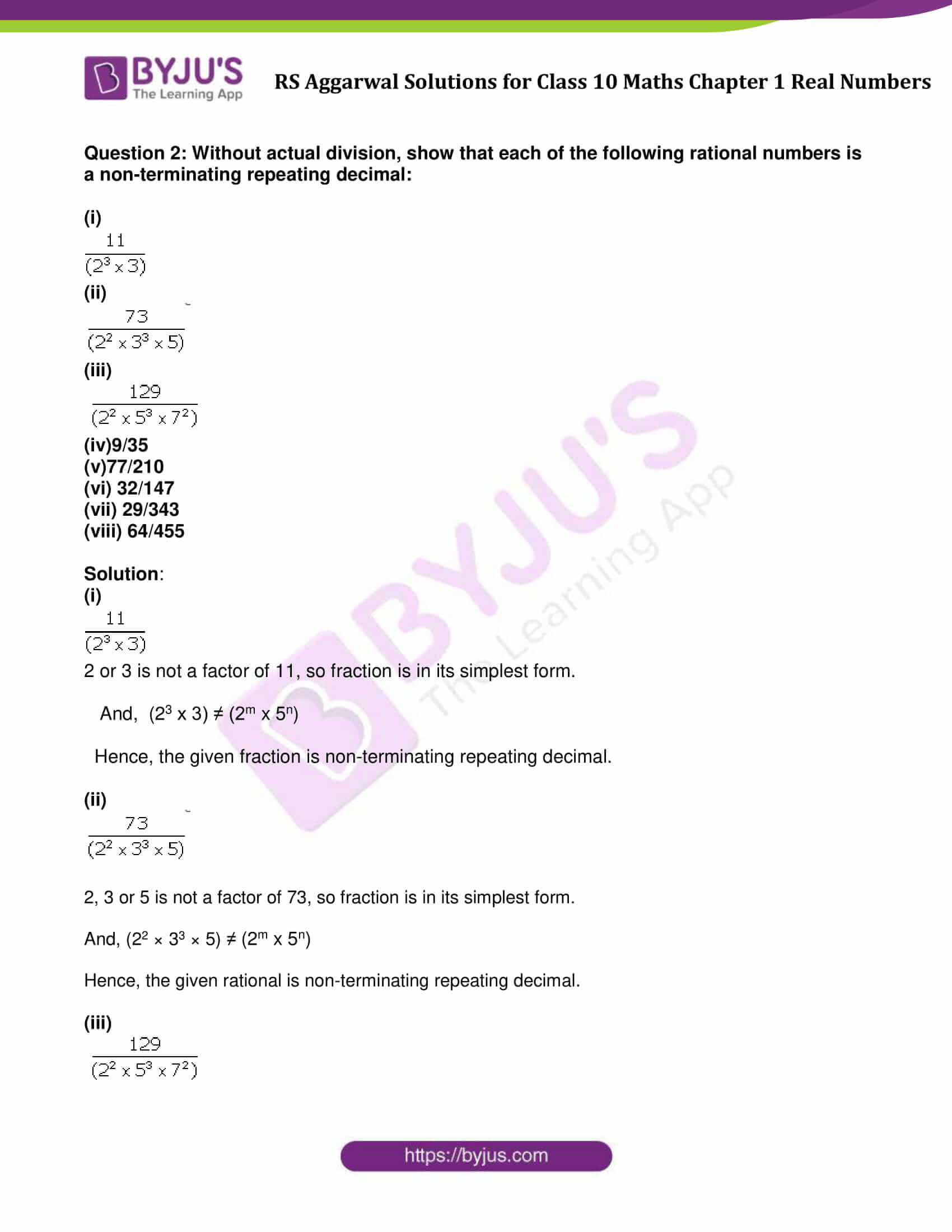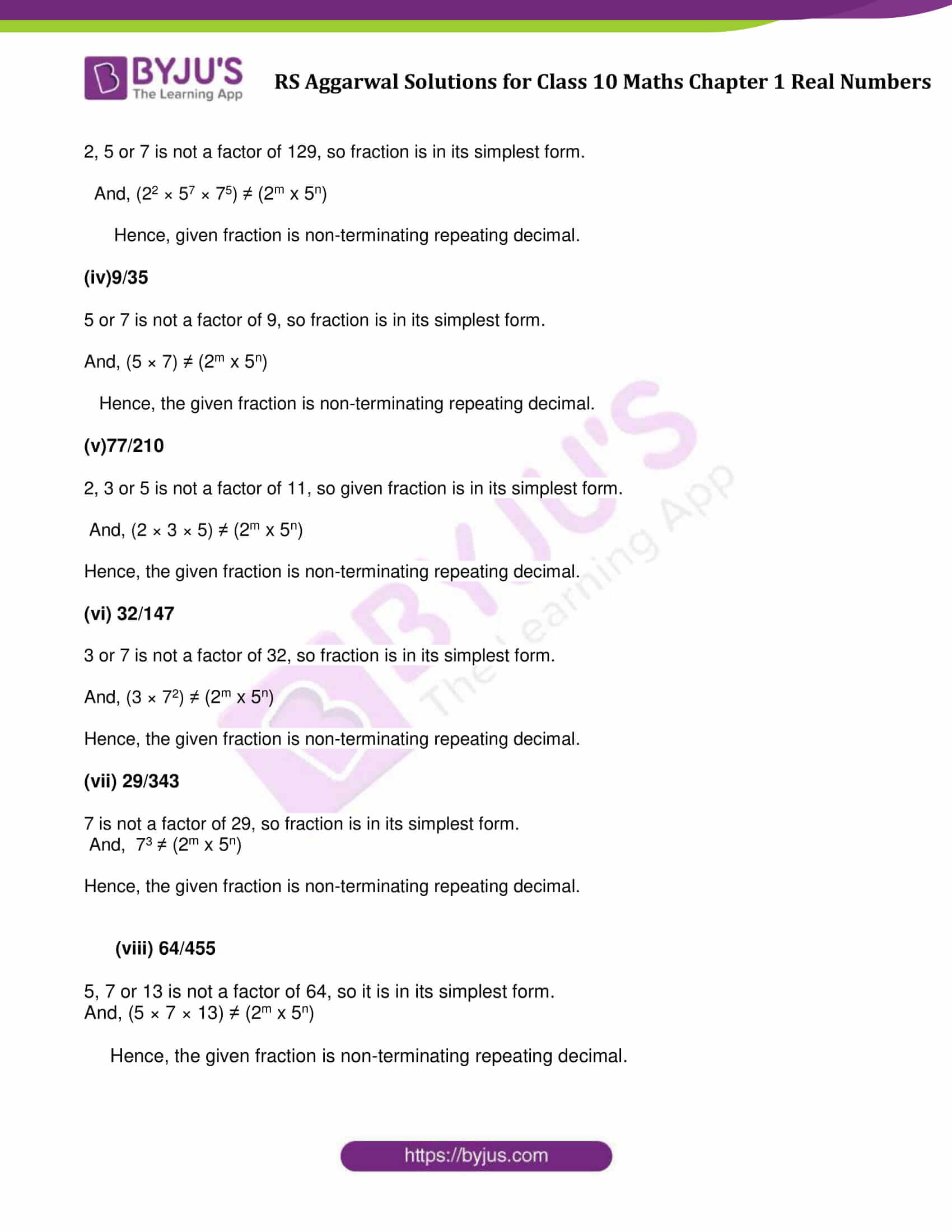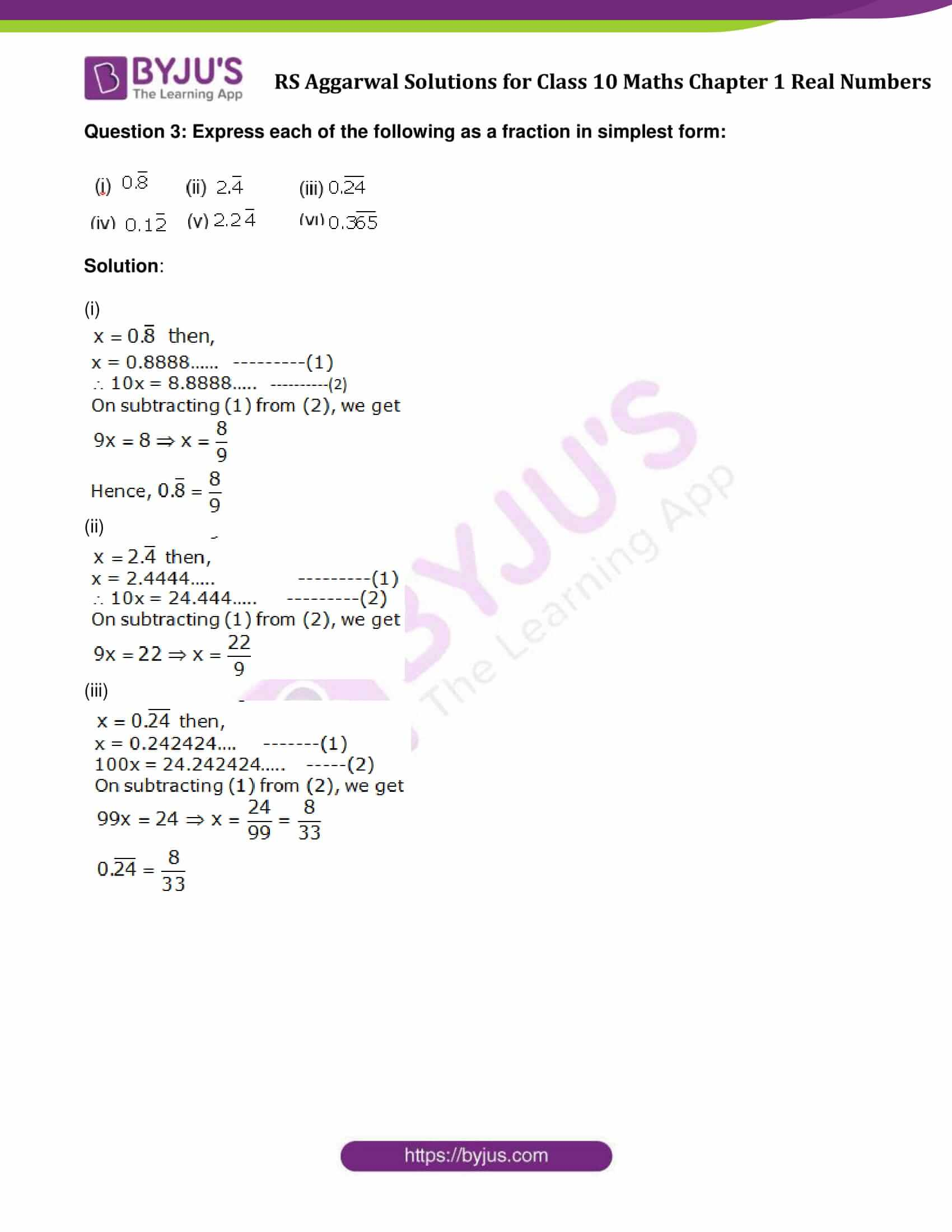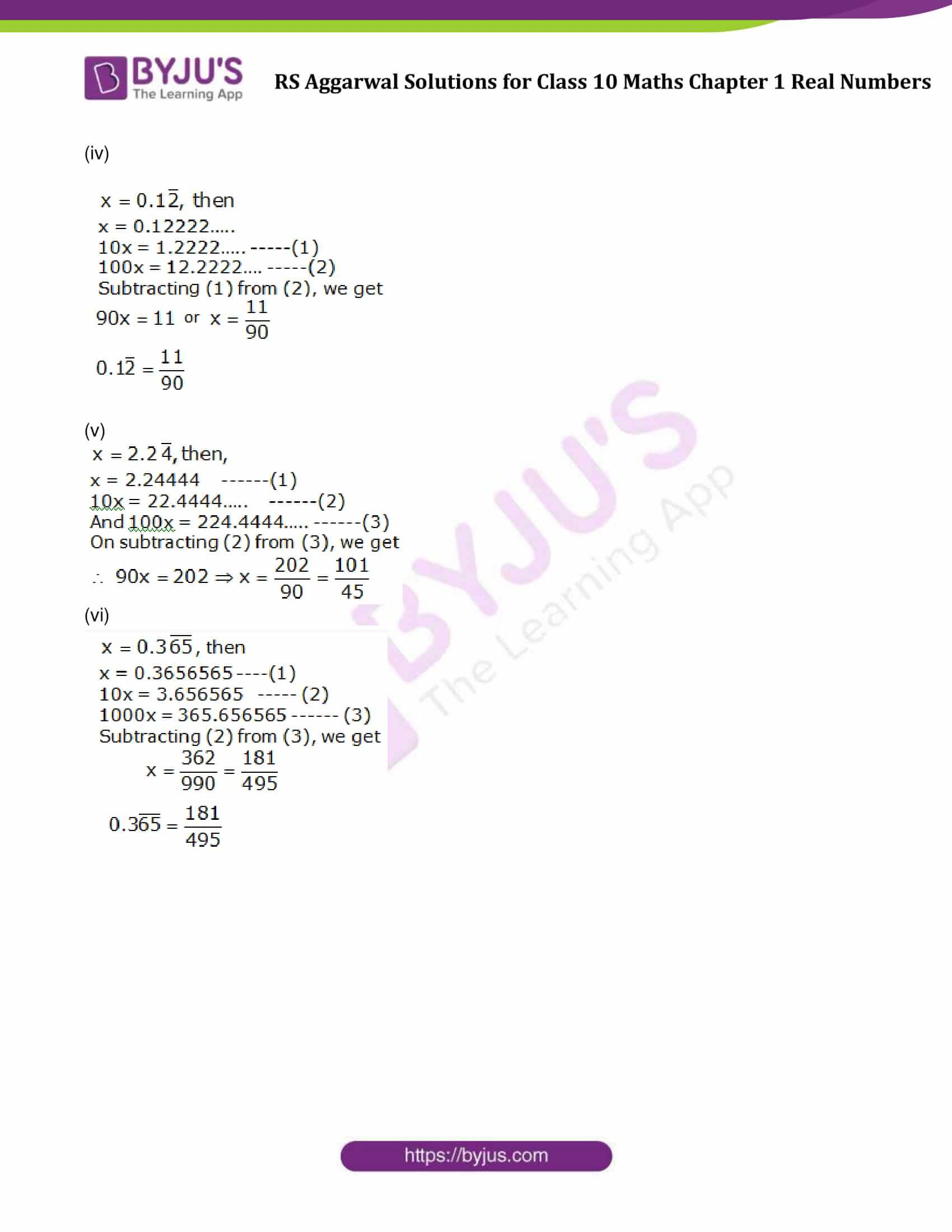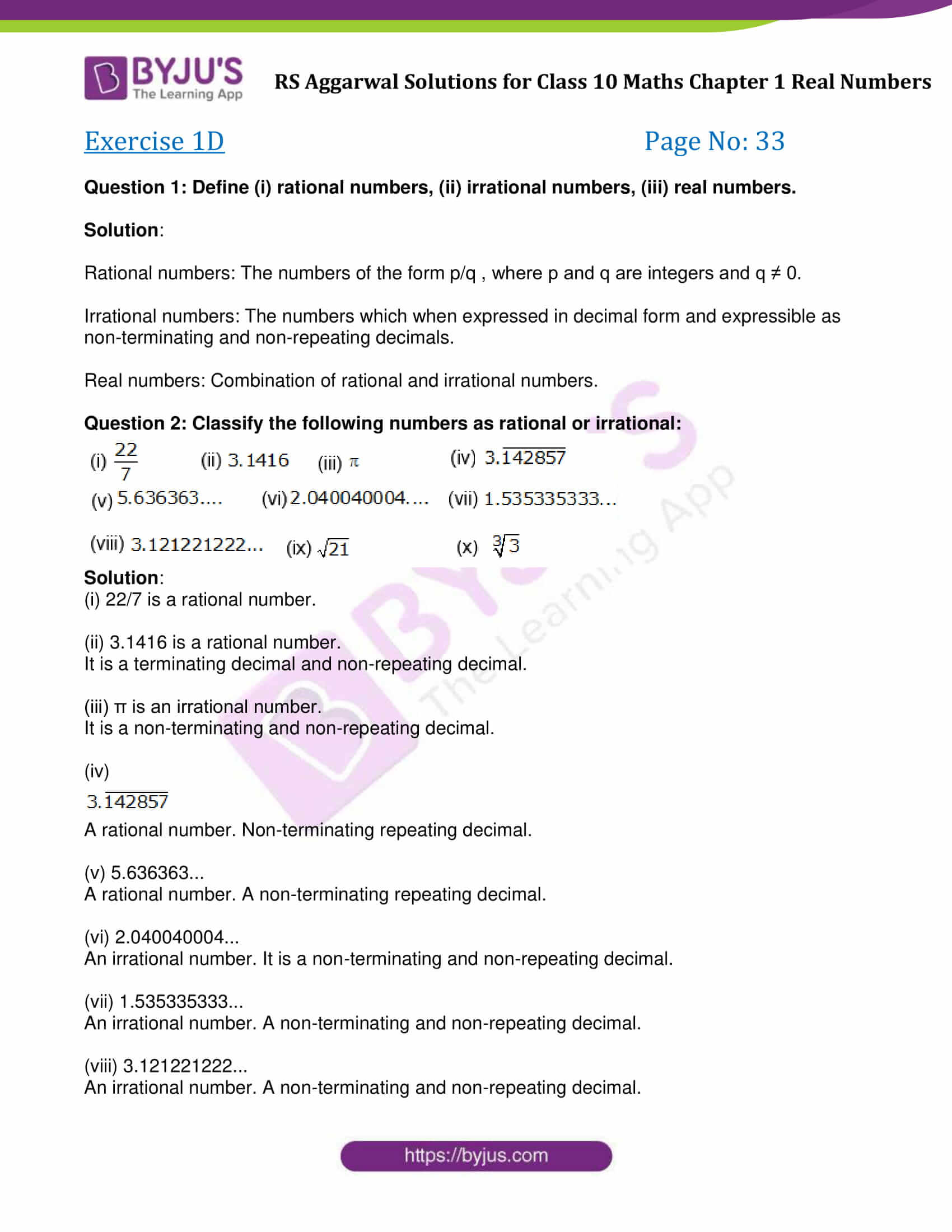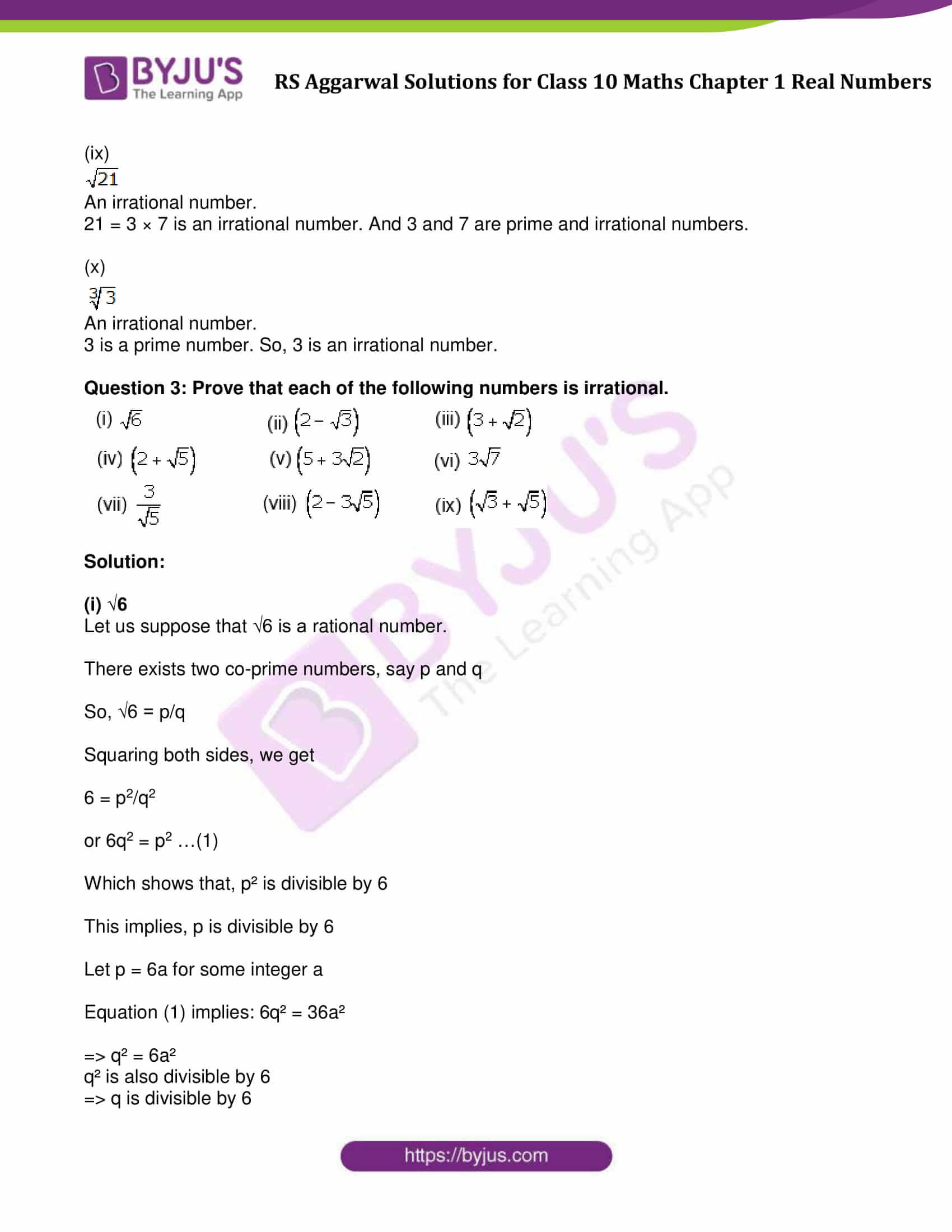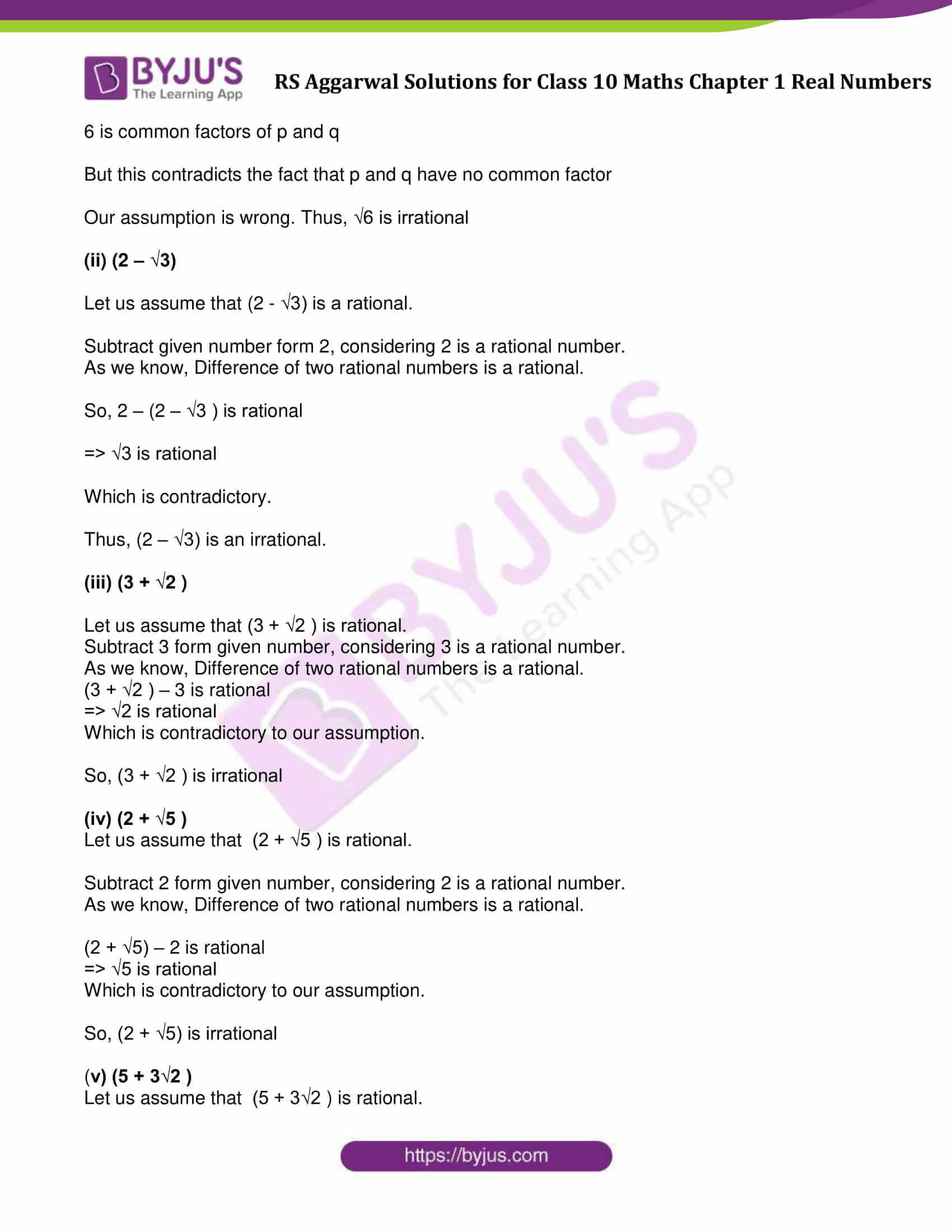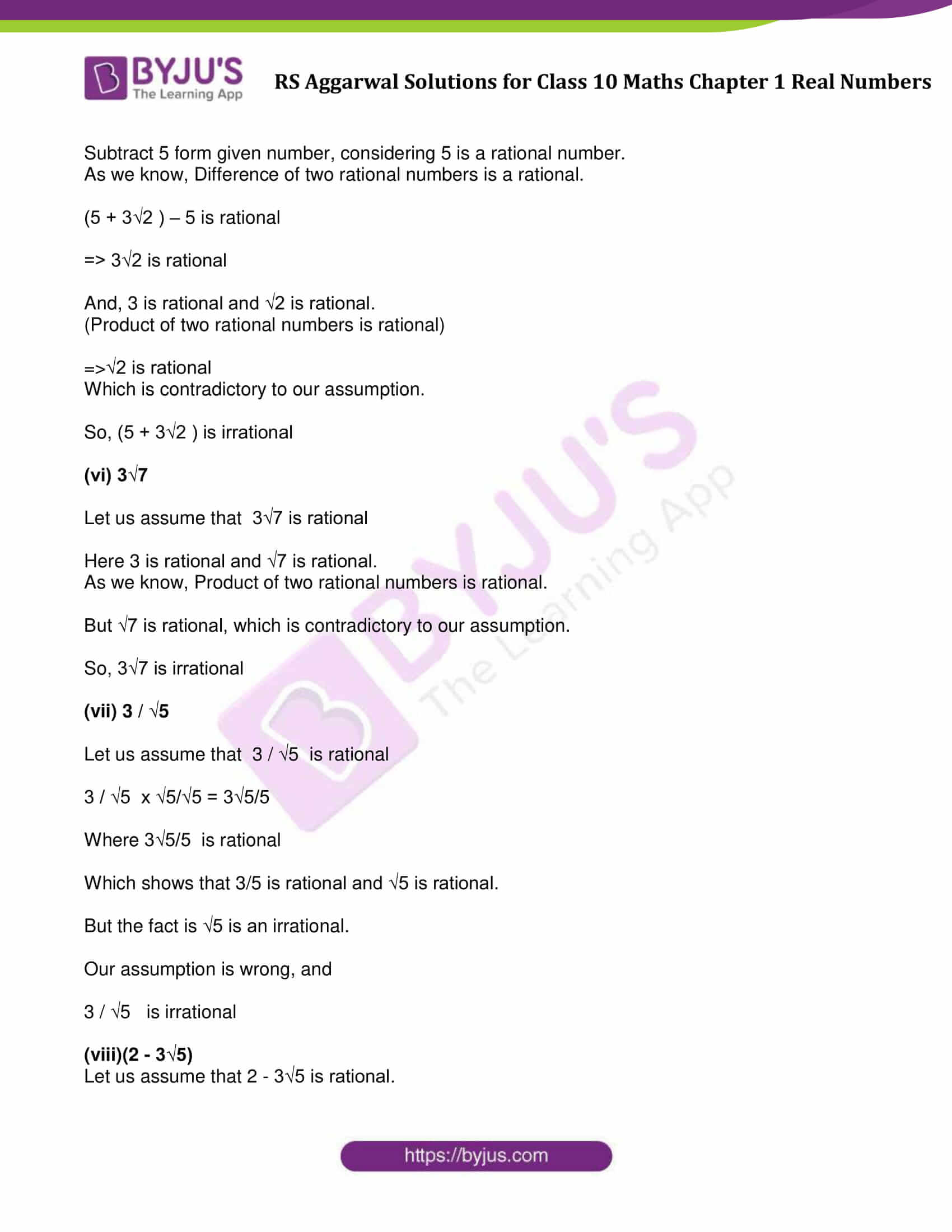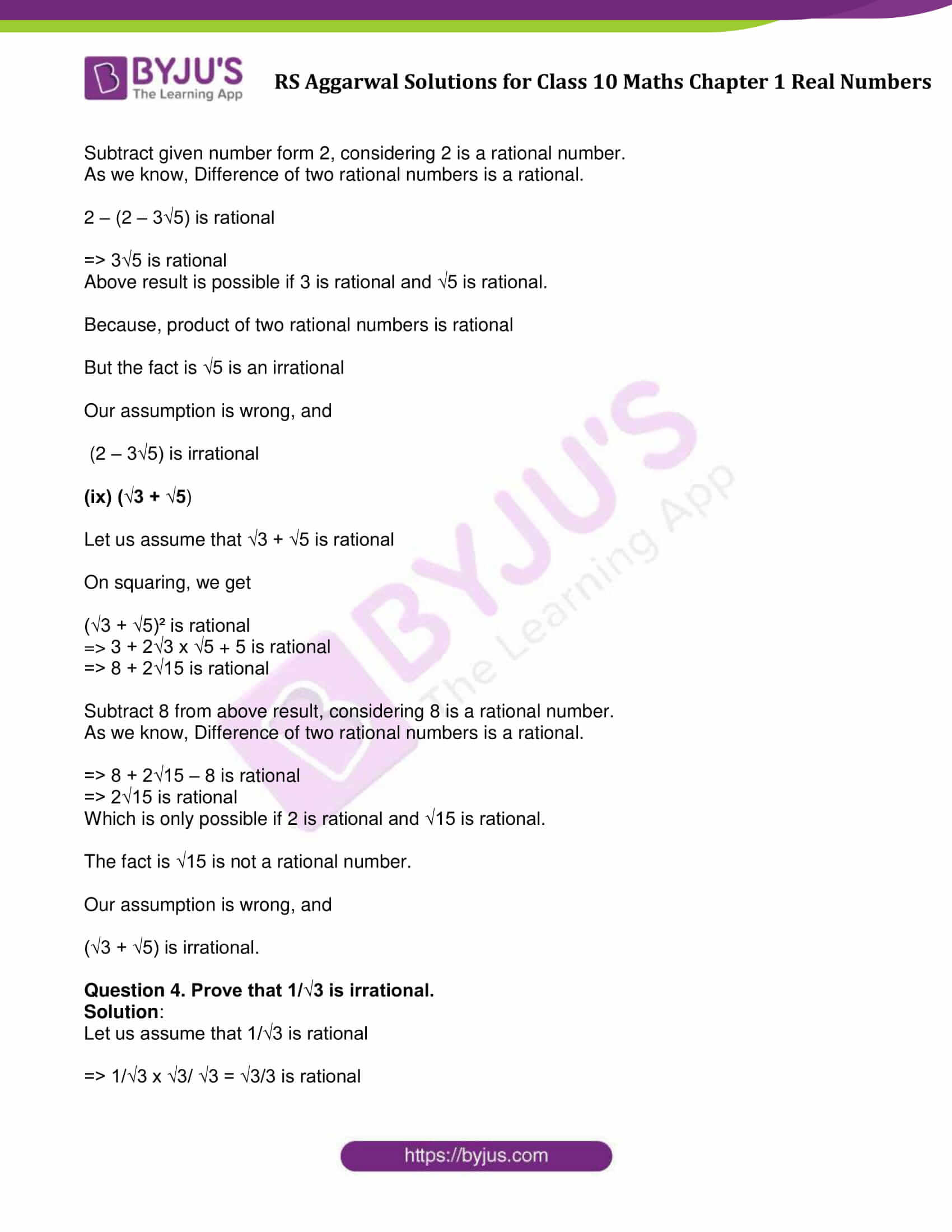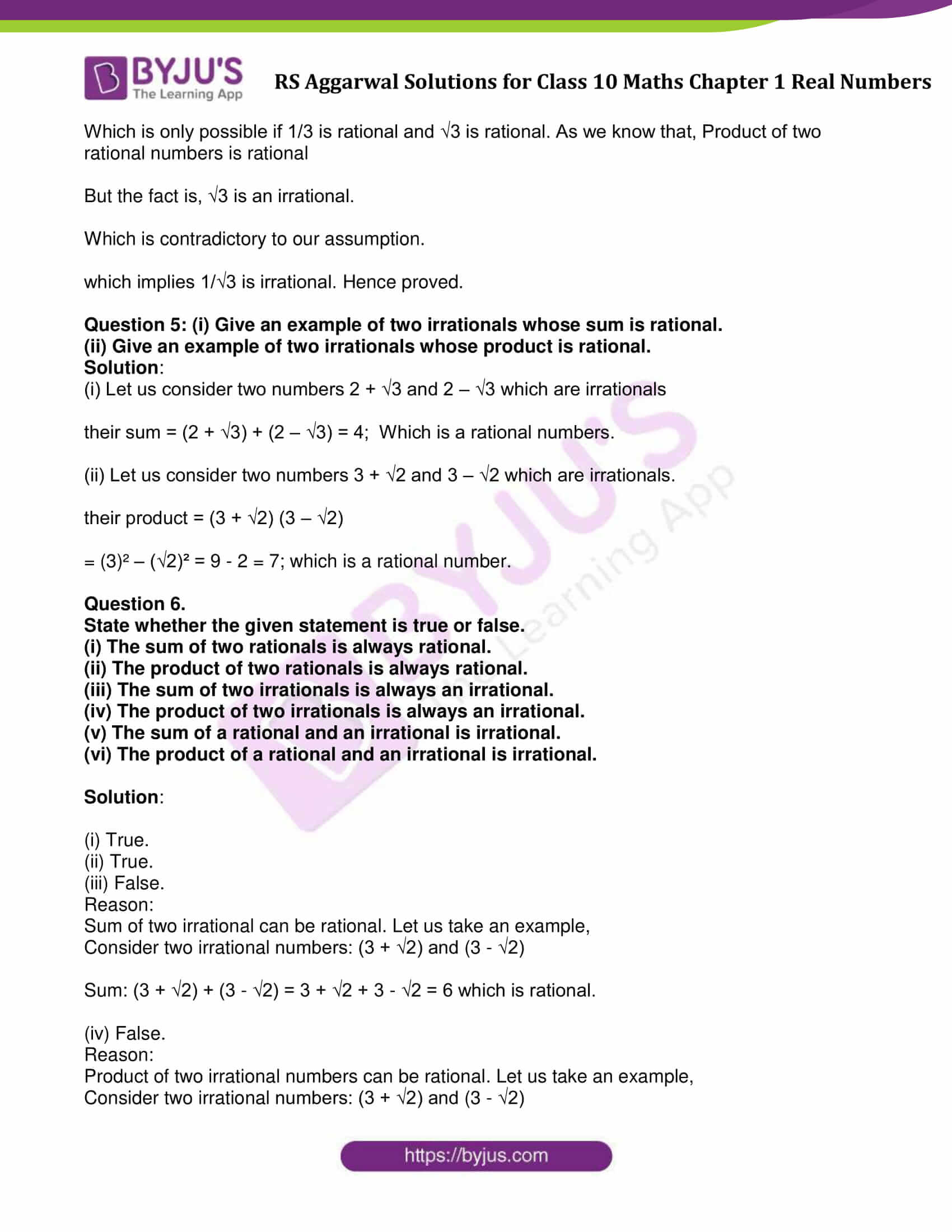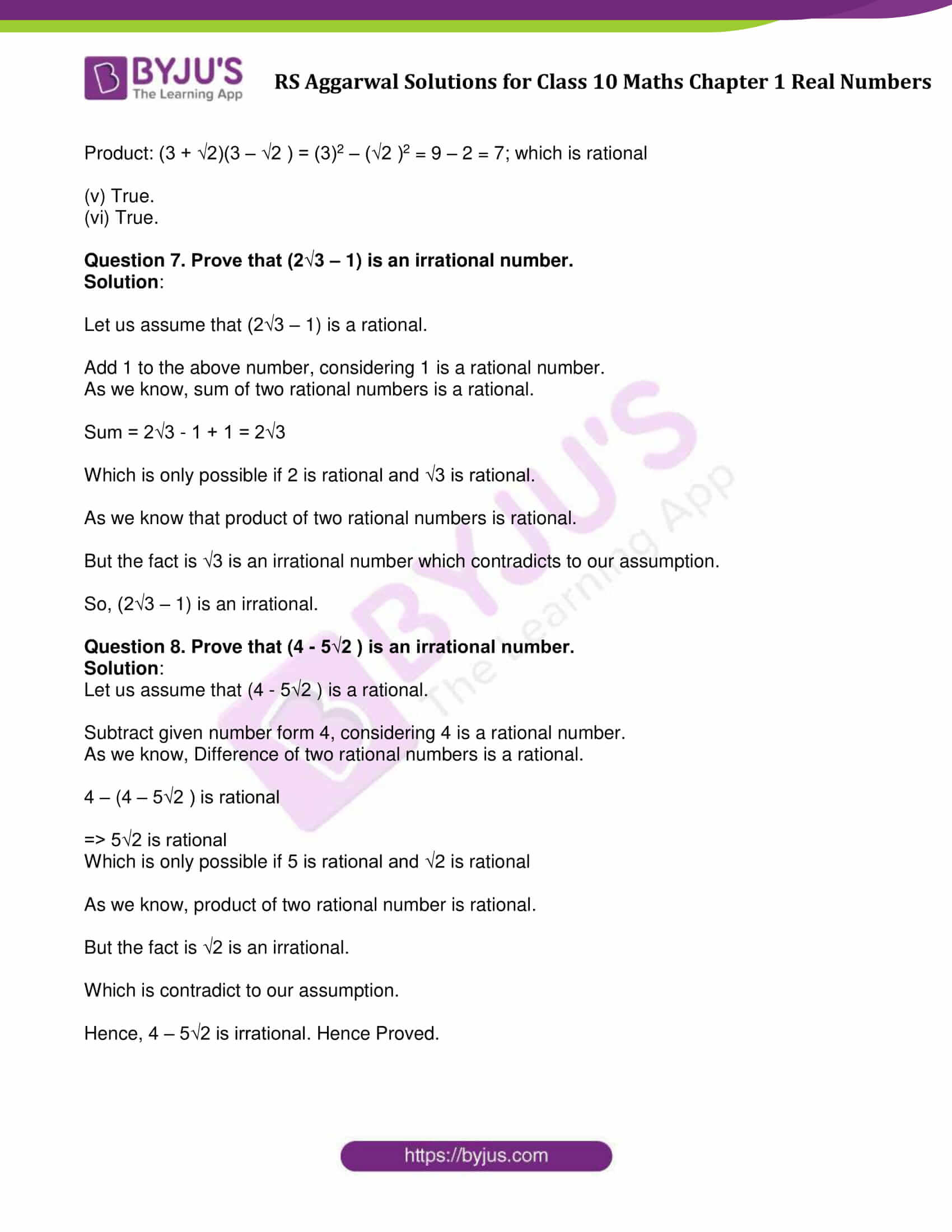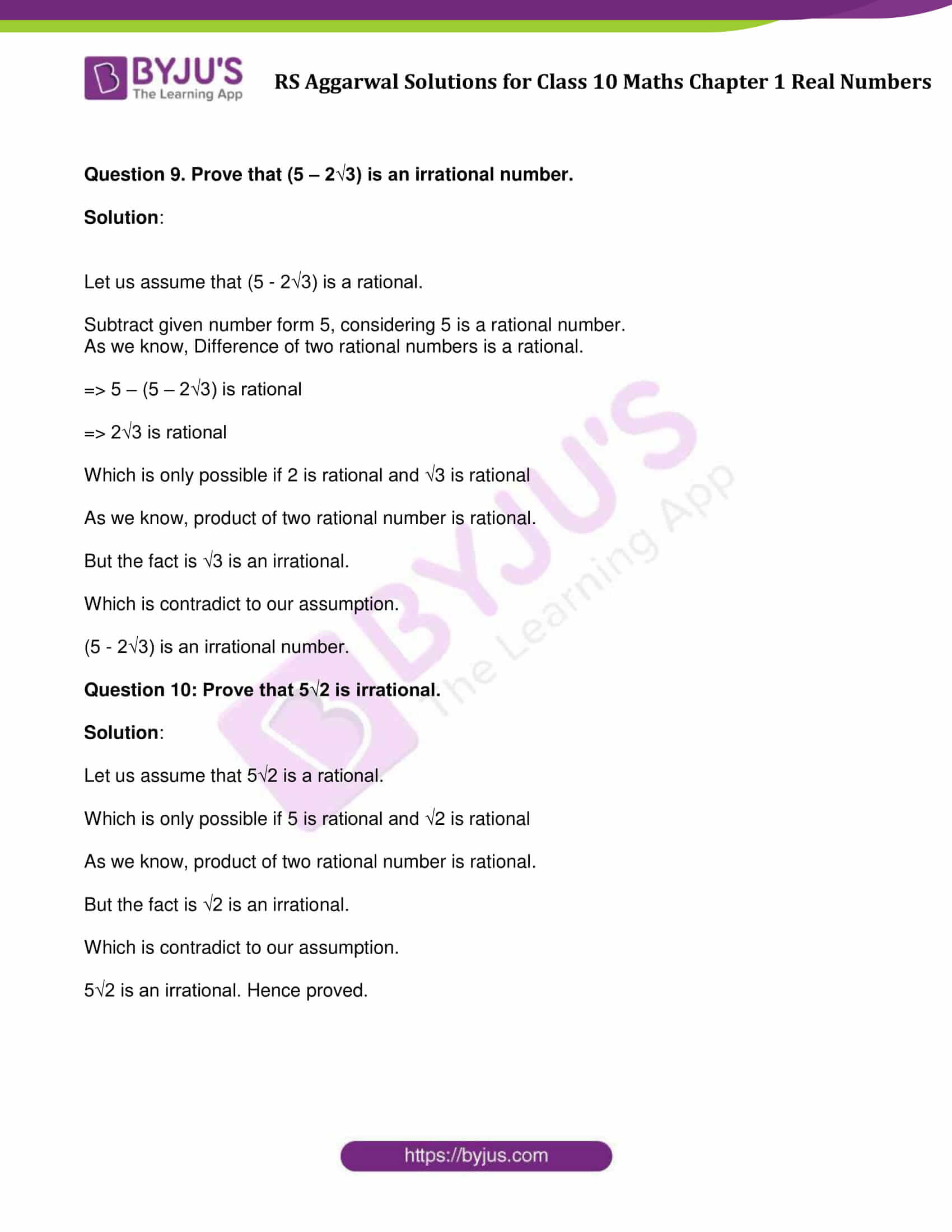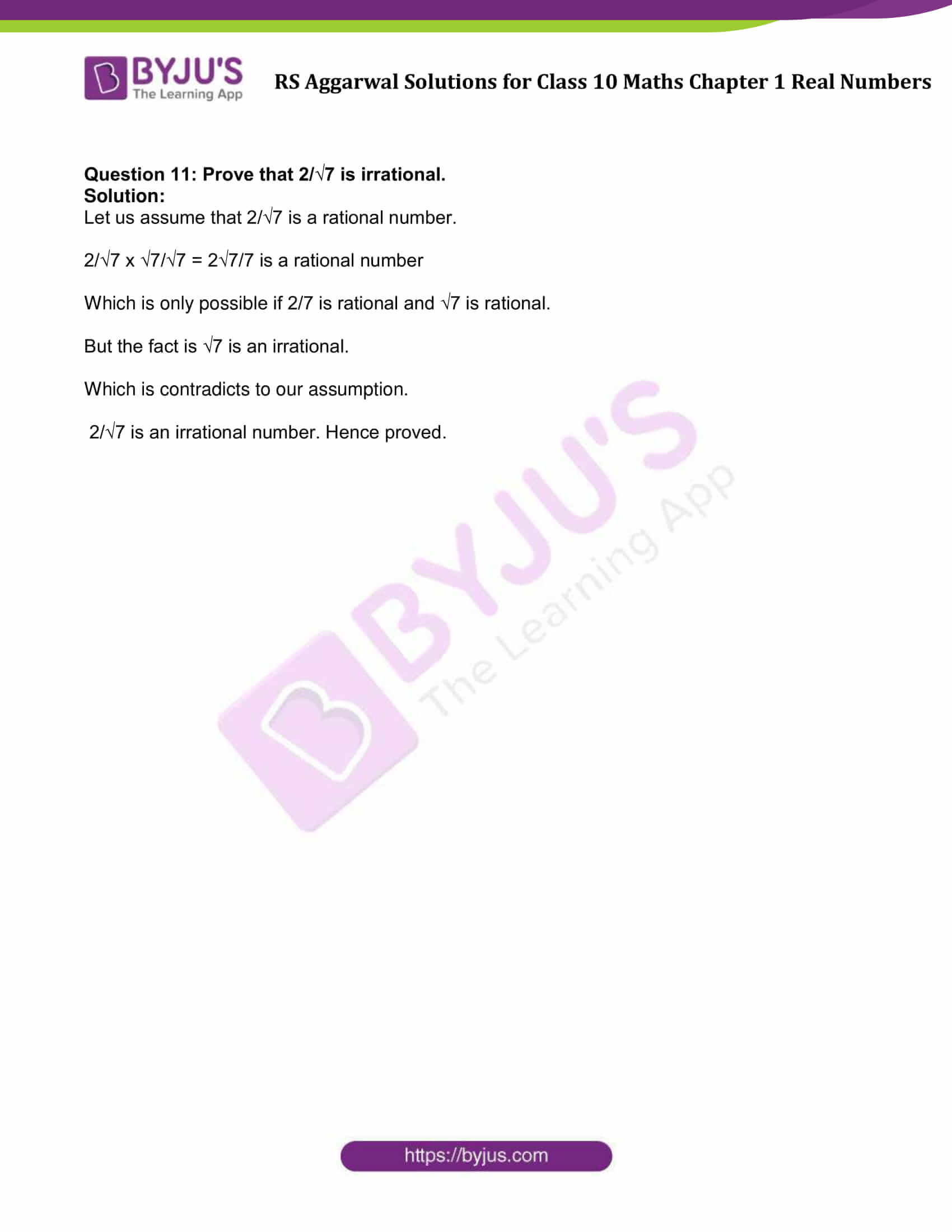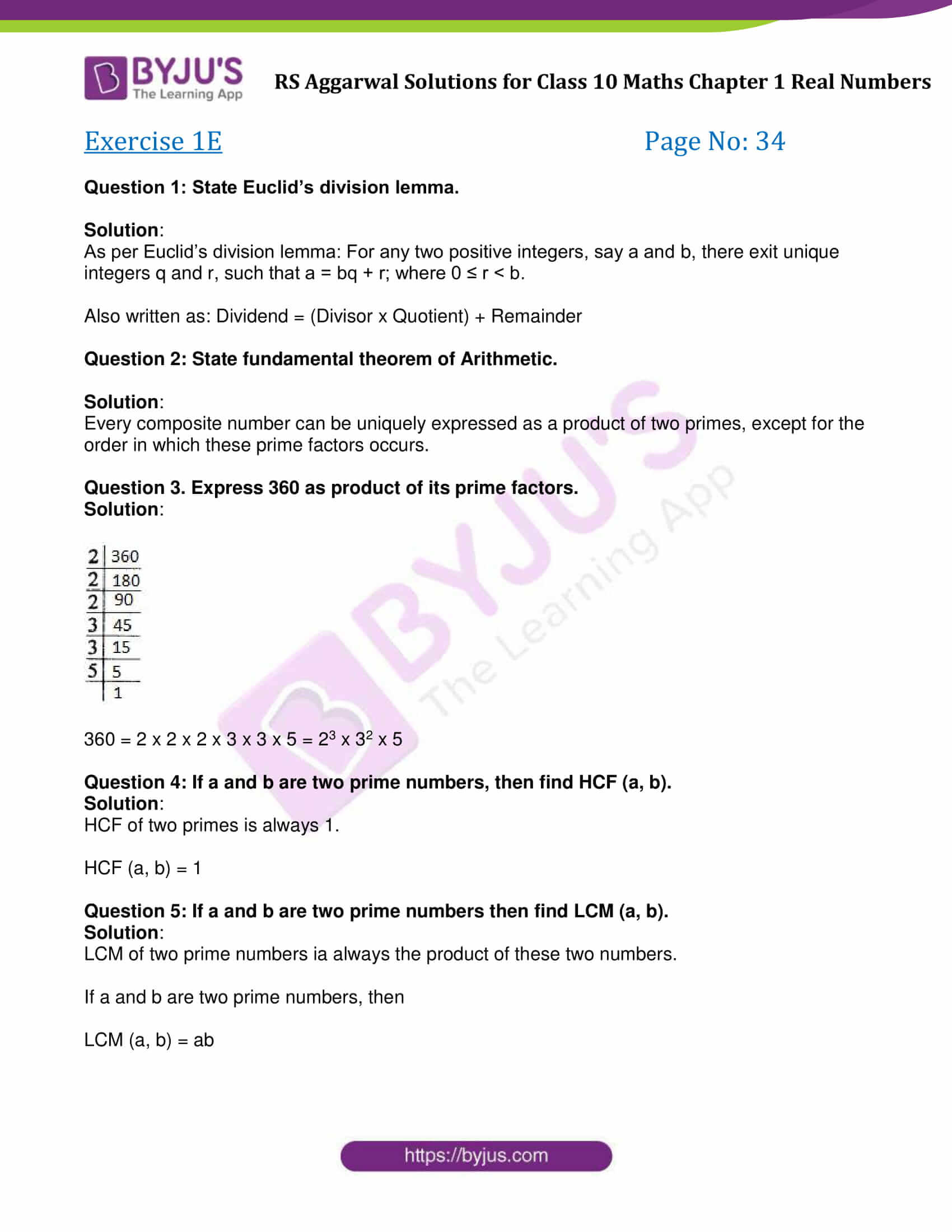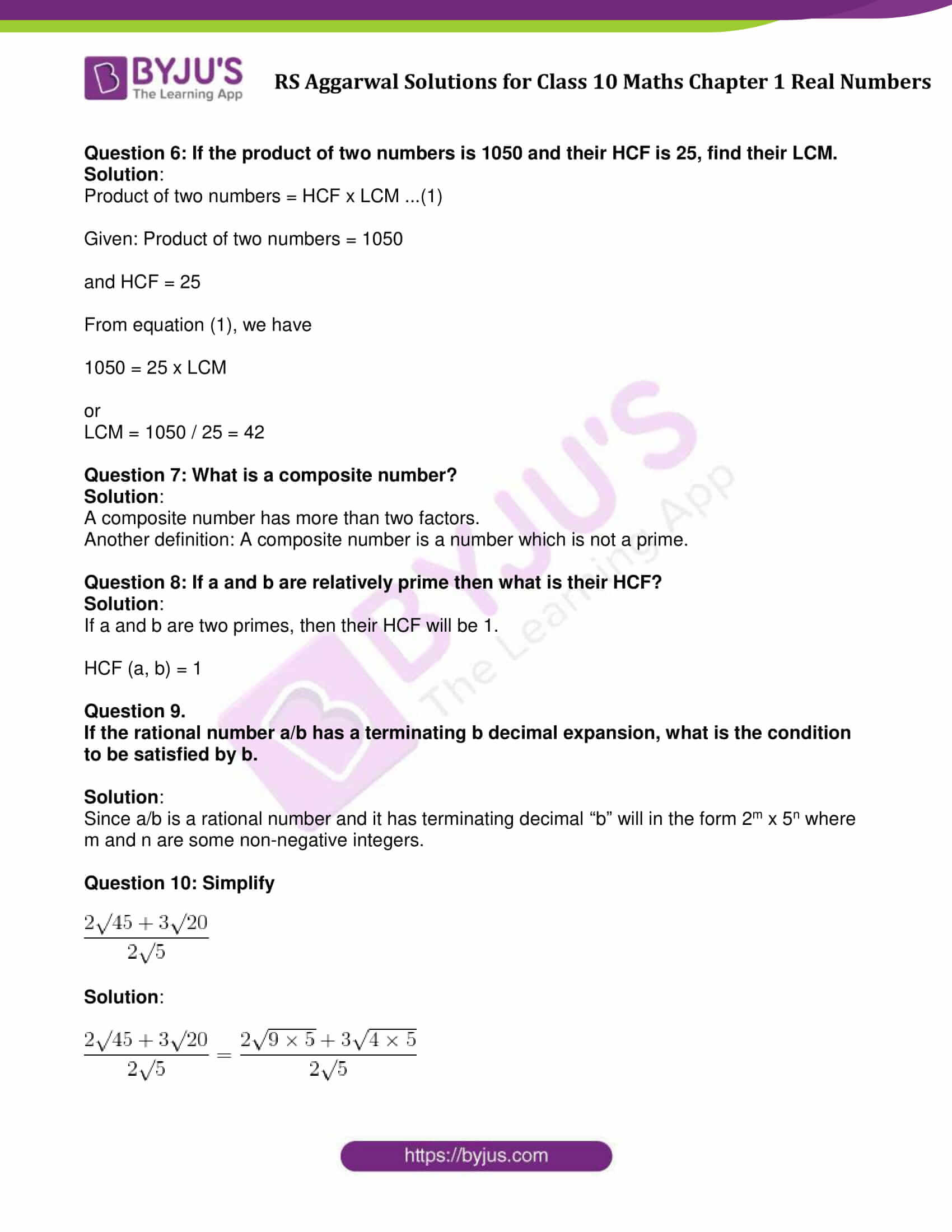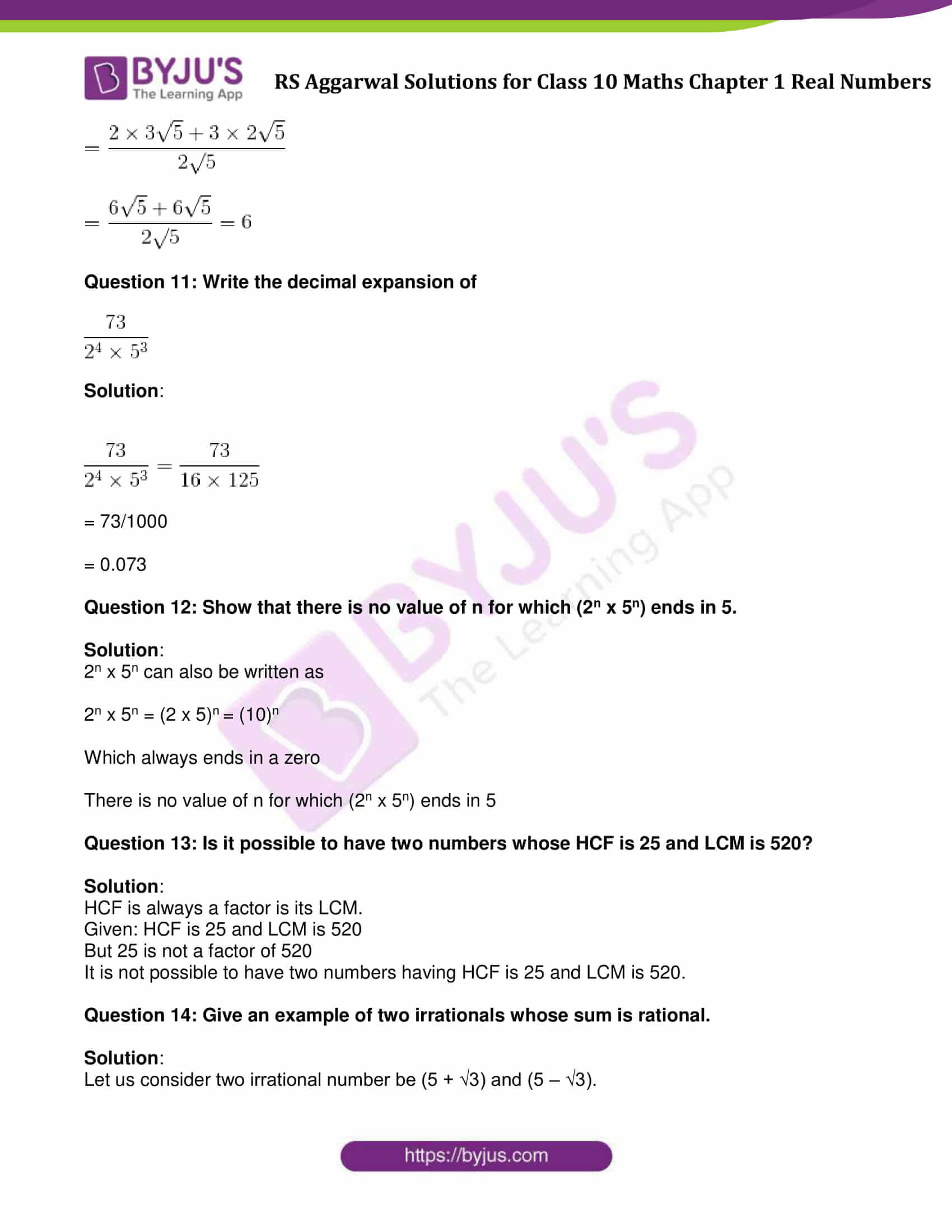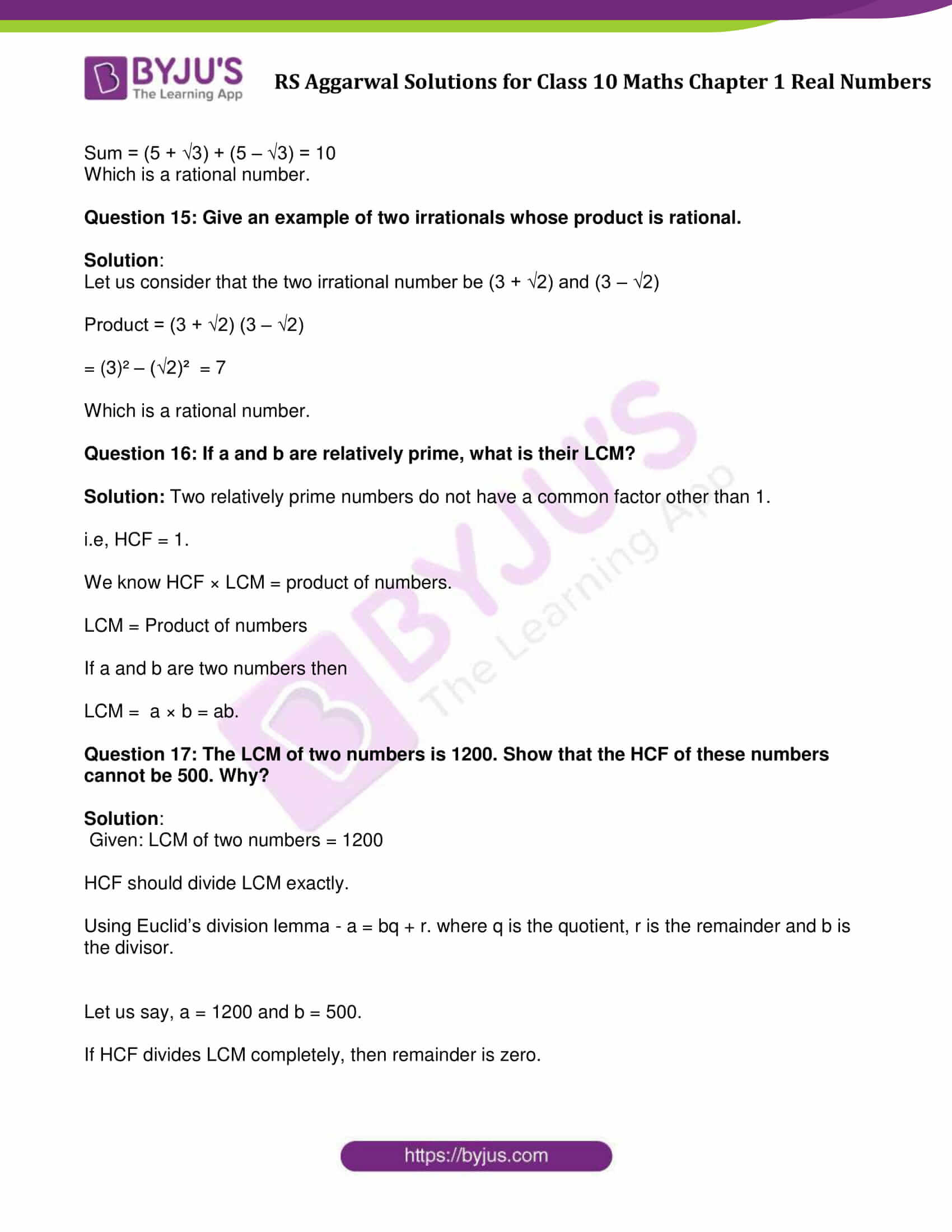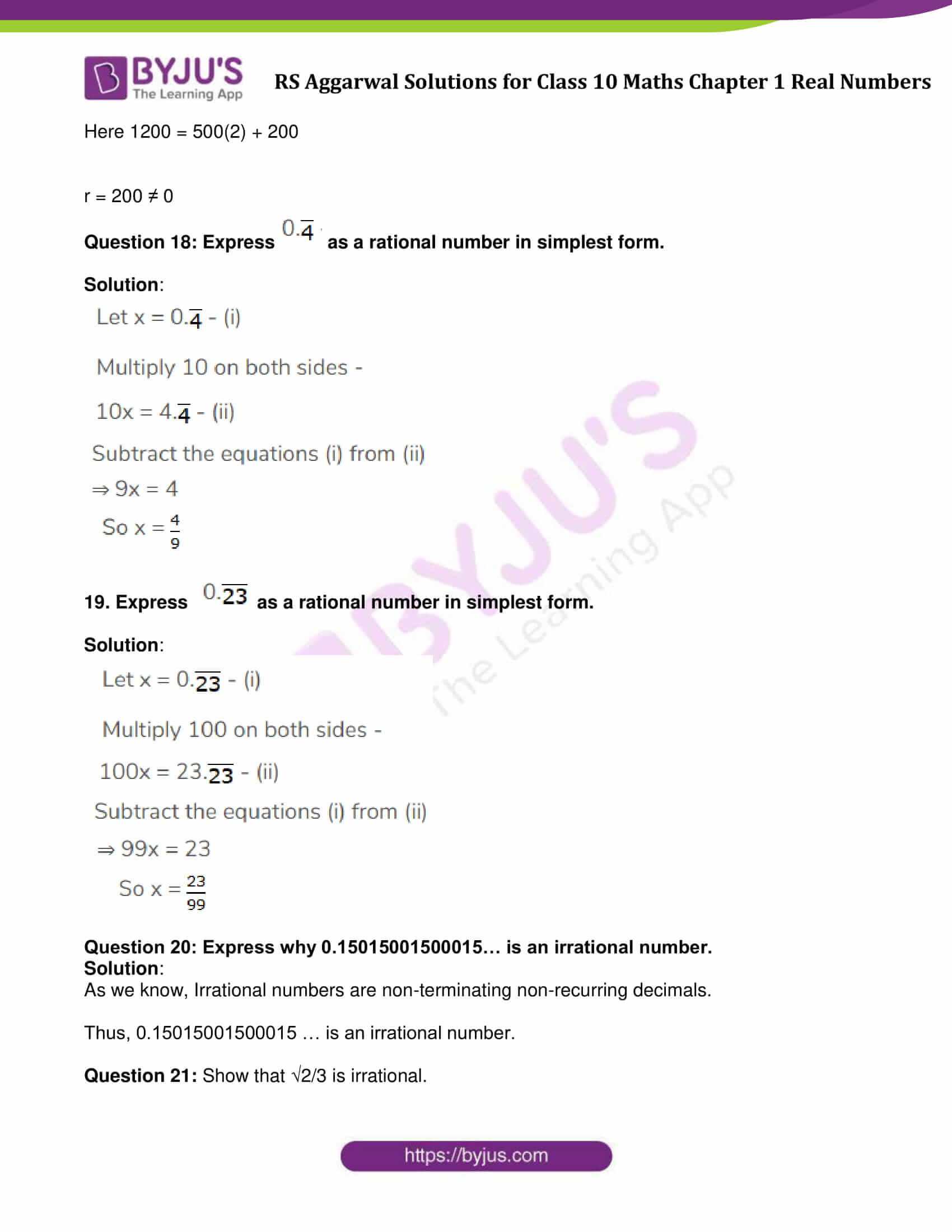### RS Aggarwal Solutions For Class 10 Maths Chapter 1 Exercises:

Get detailed solutions for all the questions listed under the below exercises:

Exercise 1A Solutions: 10 Questions (Short Answers)

Exercise 1B Solutions: 27 Questions (Short Answers)

Exercise 1C Solutions : 3 Questions (Short Answers)

Exercise 1D Solutions: 11 Questions (Short Answers)

Exercise 1E Solutions: 23 Questions (Short Answers)

## Exercise 1A Page No: 8

Question 1: What do you mean by Euclid’s division lemma?

Solution:

As per Euclid’s division lemma: For any two positive integers, say a and b, there exit unique integers q and r, such that a = bq + r; where 0 ≤ r < b.

Also written as: Dividend = (Divisor x Quotient) + Remainder

Question 2: A number when divided by 61 gives 27 as quotient and 32 as remainder. Find the number.

Solution:

Given: Divisor = 61, Quotient = 27, Remainder = 32

To Find: Dividend

As per Euclid’s lemma, we have

Dividend = (divisor x quotient) + remainder

Dividend = (61 × 27) + 32 = 1679

Therefore, dividend is 1679.

Question 3: By what number should 1365 be divided to get 31 as quotient and 32 as remainder?

Solution:

Given: Dividend = 1365, Quotient = 31 and Remainder = 32

Let us consider, Divisor as a number y.

To Find: The value of y

As per Euclid’s lemma, we have

Dividend = (divisor  \times  quotient) + remainder

After substituting the values, we get

1365 = (y × 31) + 32

1365 – 32 = 31y

1333 = 31y

or y = 1333/31 = 43

So, 1365 should be divided by 43 to get 31 as quotient and 32 as remainder.

Question 4: Using Euclid’s algorithm, find the HCF of:

(i) 405 and 2520 (ii) 504 and 1188 (iii) 960 and 1575

Solution:

(i)

Step 1: Choose bigger number: 2520 > 405

On dividing 2520 by 405, we get

Quotient = 6, remainder = 90

⇨ 2520 = (405 x 6) + 90

Step 2: On dividing 405 by 90, we get

Quotient = 4 and Remainder = 45

⇨405 = 90 x 4 + 45

Step 3: On dividing 90 by 45, we get

Quotient = 2 and Remainder = 0

⇨ 90 = 45 x 2 + 0

Step 4: Since remainder is zero, stop the process and write your answer.

Therefore, H.C.F. of 405 and 2520 is 45.

(ii)
Step 1: Choose bigger number: 1188 > 504

On dividing 1188 by 504, we get

Quotient = 2 and Remainder = 180

⇨ 1188 = 504  x 2 + 180

Step 2: on dividing 504 by 180

Quotient = 2 and Remainder = 144

⇨ 504 = 180 x 2 + 144

Step 3: on dividing 180 by 144,

Quotient = 1 and Remainder = 36

⇨ 180 = 144 x 1 + 36

Step 4: On dividing 144 by 36

Quotient = 4 and Remainder = 0

⇨ 144 = 36 x 4 + 0

Therefore, H.C.F. of 1188 and 504 is 36

(iii)

Step 1: Choose bigger number: 1575 > 960

On dividing 1575 by 960, we have

Quotient = 1, remainder = 615

⇨ 1575 = 960 x 1 + 615

Step 2: On dividing 960 by 615, we have

Quotient = 1 and Remainder = 345

⇨ 960 = 615 × 1 + 345

Step 3: On dividing 615 by 345

Quotient = 1 and Remainder = 270

⇨ 615 = 345 × 1 + 270

Step 4: On dividing 345 by 270, we have

Quotient = 1 and Remainder = 75

⇨ 345 = 270 × 1 + 75

Step 5: Dividing 270 by 75, we get

Quotient = 3, remainder =45

⇨ 270 = 75 × 3 + 45

Step 6: Dividing 75 by 45, we get

Quotient = 1, remainder = 30

⇨ 75 = 45 × 1 + 30

Step 7: Dividing 45 by 30, we get

Quotient = 1 and Remainder = 15

⇨ 45 = 30 × 1 + 15

Step 8: Dividing 30 by 15, we get

Quotient = 2 and Remainder = 0

Therefore, H.C.F. of 1575 and 960 is 15.

Question 5: Show that every positive integer is either even or odd.

Solution:

Let m be any positive integer.

If we divide m by 2, let we get q be the quotient and r be the remainder. Then by Euclid’s lemma, we have

m = 2q + r where 0 ≤ r < 2.

m = 2q + r when r = 0, 1

Which implies, m = 2q or m = 2q + 1

Case 1: When m = 2q for some integer q: which shows m is even.

Case 2: When m = 2q + 1 for some integer q: which shows m is odd.

Hence, it shows that every positive integer is either even or odd.

Question 6: Show that any positive odd integer is of the form (6m + 1) or (6m + 3) or (6m + 5), where m is some integer.

Solution:

Let s be any positive odd integer.

On dividing s by 6, let m be the quotient and r be the remainder.

By Euclid’s division lemma,

s = 6m + r, where 0 ≤ r ˂ 6

So we have, s = 6m or s = 6m + 1 or s = 6m + 2 or s = 6m + 3 or s = 6m + 4 or s = 6m + 5.

6m, 6m + 2, 6m + 4 are multiples of 2, but s is an odd integer.

Again, s = 6m + 1 or s = 6m + 3 or s = 6m + 5 are odd values of s.

Thus, any positive odd integer is of the form (6m + 1) or (6m + 3) or (6m + 5), where m is any odd integer.

Question 7: Show that any positive odd integer is of the form (4m + 1) or (4m + 3), where m is some integer.

Solution:

Let s be any positive integer.

On dividing s by 4, let m be the quotient and r be the remainder.

By Euclid’s division lemma,

s = 4m + r, where 0 ≤ r ˂ 4

So we have, s = 4m or s = 4m + 1 or s = 4m + 2 or s = 4m + 3.

Here, 4m, 4m + 2 are multiples of 2, which revert even values to s.

Again, s = 4m + 1 or s = 4m + 3 are odd values of s.

Thus, any positive odd integer is of the form (4m + 1) or (4m + 3) where s is any odd integer.

Question 8: For any positive integer n, prove that n^3 – n is divisible by 6.

Solution:

Let s be any positive integer.

On dividing s by 6, let m be the quotient and r be the remainder.

By Euclid’s division lemma,

s = 6m + r, where 0 ≤ r ˂ 6

We can say that,

Any positive integer is of the form 6m, 6m + 1, 6m + 2, 6m + 3, 6m + 4, 6m + 5 for some positive integer n.

Case 1: When n = 6m

n3 – n = (6m)3 – 6m = 216 m3 – 6m = 6m(36m2 – 1) = 6q, where q = m(36m2 -1)

n3 – n is divisible by 6

Case 2: When n = 6m + 1

n3 – n = n(n2 – 1) = n (n – 1) (n + 1) = (6m + 1) (6m) (6m + 2) = 6m(6m + 1) (6m + 2)

= 6q,

where q = m(6m + 1) (6m + 2)

n3 – n is divisible by 6

Case 3: When n = 6m + 2

n– n = n (n – 1) (n + 1) = (6m + 2) (6m + 1) (6m + 3) = (6m + 1) (36 m2 + 30m + 6)

= 6[m (36m2 + 30m + 6)] + 6 (6m2 + 5m + 1)

= 6p + 6q, where p = m (36m2 + 30m + 6) and q = 6m2 + 5m + 1

n3 – n is divisible by 6

Case 4: When n = 6m + 3

n3 – n = (6m + 3)3 – (6m + 3) = (6m + 3) [(6m + 3)2 – 1] = 6m [6m + 3)2 – 1] + 3 [(6m + 3)2 – 1]

= 6 [m [(6m + 3)2 – 1] + 6 [18m2 + 18m + 4]

= 6p + 3q,

where p = m[(6m + 3)2 – 1]

q = 18m2 + 18m + 4

n3 – n is divisible by 6

Case 5: When n = 6m + 4

n3 – n = (6m + 4)3 – (6m + 4) = (6m + 4) [(6m + 4)2 – 1]

= 6m [(6m + 4)2 – 1] + 4 [(6m + 4)2 – 1]

= 6m [(6m + 4)2 – 1] + 4 [36m2 + 48m + 16 – 1]

= 6m [(6m + 4)2 – 1] + 12 [12m2 + 16m + 5]

= 6p + 6q,

where p = m [(6m + 4)2 – 1]

q = 2 (12 m2 + 16m + 5)

n3 – n is divisible by 6

Case 6: When n = 6m + 5

n3 – n = (6m + 5) [(6m + 5)2 – 1] = 6m [(6m + 5)2 – 1] + 5 [(6m + 5)2 – 1]

= 6p + 30q

= 6 (p + 5q),

where p = m [(6m + 5)2 – 1]

q = 6m2 + 10m + 4

n3 – n is divisible by 6

Therefore, n3 – n is divisible by 6, for any positive integer n.

Question 9: Prove that if x and y are both odd positive integers then x^2 + y^2 is even but not divisible by 4.

Solution:

Let us consider two odd positive numbers be x and y where

x = 2p + 1 and y = 2q + 1

From question,

x2 + y2 = (2p + 1)2 +(2q + 1)2

= 4p2 + 4p + 1 + 4q2 + 4q + 1

= 4p2 + 4q2 + 4p + 4q + 2

= 4 (p2 + q2 + p + q) + 2

Form above result, we can conclude that x and y are odd positive integer, then x2 + y2 is even but not divisible by four.

Question 10: Use Euclid’s algorithm to find HCF of 1190 and 1145. Express the HCF in the form 1190m + 1445n.

Solution:

Here 1145 > 1190

Use Euclid’s algorithm

1445 =1190 x 1 + 255

1190 = 255 x 4 +170

255 = 170 x 1 + 85

170 = 85 x 2 + 0

Remainder is zero. Stop the process.

This implies, HCF = 85

Now, Express the HCF in the form 1190m + 1445n.

85 = 255 – 170

= (1445 – 1190) – (1190 – 255 x 4)

= 1445 – 1190 – 1190 + 255 x 4

= 1445 – 1190 x 2 + 1445 x 4 – 1190 x 4

= 1445 x 5 – 1190 x 6

= 1190 x (-6) + 1445 x 5

choose m = -6 and n = 5

= 1190 m + 1445n

## Exercise 1B Page No: 15

Question 1: Using prime factorization, find the HCF and LCM of:

(i) 36, 84 (ii) 23, 31 (iii) 96, 404

(iv) 144,198 (v) 396, 1080 (vi) 1152, 1664

In each case, verify that:

HCF x LCM = Product of given numbers

Solution:

(i)Find Prime factors of 36 and 84

36 = 2 x 2 x 3 x 3

84 = 2 x 2 x 3 x 7

LCM (36, 84) = 2 x 2 x 3 x 3 x 7 = 252

HCF (36, 84) = 2 x 2 x 3 = 12

Verification:

HCF x LCM = 12 x 252 = 3024

Product of given numbers = 36 x 84 = 3024

⇨ HCF x LCM = Product of given numbers

Verified.

(ii)Find Prime factors of 23 and 31

23 and 31 are prime numbers.

LCM (23 and 31) = 23 x 31 = 713

HCF (23 and 31) = 1

Verification:

HCF x LCM = 1 x 713 = 713

Product of given numbers = 23 x 31 = 713

⇨ HCF x LCM = Product of given numbers

Verified.

(iii)Find Prime factors of 96 and 404

96 = 2 x 2 x 2 x 2 x 2 x 3

404 = 2 x 2 x 101

LCM (96 and 404) = 25 × 3 × 101 = 9696

HCF (96 and 404) = 22 = 4

Verification:

HCF x LCM = 9696 x 4 = 38784

Product of given numbers = 96 x 404 = 38784

⇨ HCF x LCM = Product of given numbers

Verified.

(iv) Find Prime factors of 144 and 198

144 = 24 × 32

198 = 2 × 32 × 11

LCM (144, 198) = 24 × 32 × 11 = 1584

HCF (144, 198) = 2 x 32 = 18

Verification:

HCF x LCM = 1584 x 18 = 28512

Product of given numbers = 144 x 198 = 28512

⇨ HCF x LCM = Product of given numbers

Verified.

(v)Find Prime factors of 396 and 1080

396 = 22 × 32 × 11

1080 = 23 × 33 x 5

LCM (396, 1080) = 23 × 33 x 5 x 11 = 11880

HCF (396, 1080) = 22 × 32 = 36

Verification:

HCF x LCM = 11880 x 36 = 427680

Product of given numbers = 396 x 1080 = 427680

⇨ HCF x LCM = Product of given numbers

Verified.

(vi) Find Prime factors of 1152 and 1664

1152 = 27 × 32

1664 = 27 × 13

LCM (1152, 1664) = 27 × 32 × 13 = 14976

HCF (1152, 1664) = 27 = 128

Verification:

HCF x LCM = 14976 x 128 = 1916928

Product of given numbers = 1152 x 1664 = 1916928

⇨ HCF x LCM = Product of given numbers

Verified.

Question 2: Using prime factorization, find the HCF and LCM of:

(i) 8, 9, 25
(ii) 12, 15, 21
(iii) 17, 23, 29
(iv) 24, 36, 40
(v) 30, 72, 432
(vi) 21, 28, 36, 45

Solution :

(i) Prime factors of 8, 9, 25

8 = 2 × 2 × 2 = 23

9 = 3 × 3 = 32

25 = 5 × 5 = 52

HCF(8, 9, 25) = 1

LCM (8, 9, 25) = 23 x 32 x 52 = 1800

(ii) Prime factors of 12, 15, 21

12 = 2 × 2 × 3 = 22 × 3

15 = 3 × 5

21 = 3 × 7

HCF(12, 15, 21) = 3

LCM (12, 15, 21) = 22 × 3× 5 × 7 = 420

(iii) Prime factors of 17, 23, 29

17, 23 and 29 are prime numbers.

HCF(17, 23, 29) = 1

LCM (17, 23, 29) = 17 x 23 x 29 = 11339

(iii) Prime factors of 17, 23, 29

17, 23 and 29 are prime numbers.

HCF(17, 23, 29) = 1

LCM (17, 23, 29) = 17 x 23 x 29 = 11339

(iv) Prime factors of 24, 36, 40

24 = 2 × 2 × 2 × 3 = 23 × 3

36 = 2 × 2 × 3 × 3 = 22 × 32

40 = 2 × 2 × 2 × 5 = 23 × 5

HCF(24, 36, 40) = 22 = 4

LCM (24, 36, 40) = 22 × 32× 5 = 360

(v) Prime factors of 30, 72, 432

30 = 2 × 3 × 5

72 = 2 × 2 × 2 × 3 × 3 = 23 × 32

432 = 2 × 2 × 2 × 2 × 3 × 3 × 3 = 24 × 33

HCF(30, 72, 432) = 2 x 3 = 6

LCM (30, 72, 432) = 24 × 33× 5 = 2160

(vi) Prime factors of 21, 28, 36, 45

21 = 3 × 7

28 = 2 × 2 × 7 = 22 × 7

36 = 2 × 2 × 3 × 3 = 22 × 32

45 = 5 × 3 × 3 = 5 × 32

HCF(21, 28, 36, 45) = 1

LCM (21, 28, 36, 45) = 22 × 32 x 5 × 7 = 1260

Question 3: The HCF of two numbers is 23 and their LCM is 1449. If one of the numbers is 161, find the other.

Solution:

Given: HCF = 23, LCM = 1449 and one number = 161

Let the other number be m.

We know, product of two numbers = HCF x LCM

161 x m = 23 × 1449

Simplify above expression and find the value of m:

m = (23 x 1449)/161 = 207

Therefore, the second number is 207

Question 4:

The HCF of two numbers is 145 and their LCM is 2175. If one of the numbers is 725, find the other.

Solution:

Given: HCF = 145

LCM = 2175

One of the two numbers = 725

Let another number be m.

To Find: The value of m

We know, product of two numbers = HCF × LCM

Substitute the values, we get

725 x m = 145 x 2175

m = (145 x 2175)/725 = 435

Question 5: The HCF of two numbers is 18 and their product is 12960. Find their LCM.

Solution:

Given: HCF = 18

Product of two numbers = 12960

To find: LCM of two numbers

We know, product of two numbers = HCF x LCM

12960 = 18 x LCM

LCM = 720

Question 6: Is it possible to have two numbers whose HCF is 18 and LCM is 760? Give reason.

Solution:

No, it is not possible.

Reason:

HCF must be a factor of LCM. Here 18 is not factor of 760.

Question 7: Find the simplest form of:

(i) 69/92
(ii) 473/645
(iii) 1095/1168
(iv) 368/496

Solution:

(i) 69/92
$\frac{69}{92} = \frac{3\times23}{2\times2\times23} = \frac{3}{4}$

(ii) 473/645
$\frac{473}{645} = \frac{11\times43}{3\times5\times43} = \frac{11}{15}$

(iii) 1095/1168
$\frac{1095}{1168} = \frac{3\times5\times73}{2^{4}\times73} = \frac{15}{16}$

(iv) 368/496
$\frac{368}{496} = \frac{2\times2\times2\times2\times23}{2\times2\times2\times2\times31} = \frac{23}{31}$

Question 8:

Find the largest number which divides 438 and 606, leaving remainder 6 in each case.

Solution:

The largest number divides 438 and 606, leaving remainder 6 is the largest number divides 432 (i.e. 438 – 6 = 432) and 600 (i.e. 606 – 6 = 600)

HCF of 432 and 600 gives the required number.

To find: HCF of 432 and 600

Find HCF of 432 and 600 using prime factorization

432 = 24 × 33

600 = 23 × 3 × 52

HCF = 23 × 3 = 24

Therefore, the required number is 24.

Question 9:

Find the largest number which divides 320 and 457, leaving remainders 5 and 7 respectively.

Solution:

Step 1: Subtract 5 from 320 and 7 form 457, we get

320 – 5 = 315

457 – 7 = 450

HCF of 315 and 450 gives the required number.

Using prime factorization:

315 = 3 × 3 × 5 × 7

= 32 × 5 × 7

450 = 2 × 3 × 3 × 5 × 5

= 2 × 32 × 52

HCF of 315 and 450 is 32× 5 = 9 × 5 = 45

The required number is 45.

Question 10: Find the least number which when divided by 35, 56 and 91 leaves the same remainder 7 in each case.

Solution:

The required number is determine with the help of LCM of 35, 56 and 91.

Using prime factorization, find the LCM:

35 = 5 x 7

56 = 2 x 2 x 2 x 7

91 = 7 x 13

LCM (35, 56 and 91) = 23 x 5 x 7 x 13 = 3640

Least number which when divided by 35, 56 and 91 leaves the same remainder 7 is 3640 + 7 = 3647.

Question 11: Find the smallest number which when divided by 28 and 32 leaves remainders 8 and 12 respectively.

Solution:

The required number is determine with the help of LCM of 28 and 32.

Using prime factorization, find the LCM:

28 = 2 x 2 x 7

32 = 2 x 2 x 2 x 2x 2

LCM = 224

Find the smallest number which when divided by 28 and 32 leaves remainders 8 and 12 is 224 – (8 + 12) = 204

Question 12: Find the smallest number which when increased by 17 is exactly divisible by both 468 and 520.

Solution:

The required number is determine with the help of LCM of 468 and 520.

Using prime factorization, find the LCM:

468 = 22 × 32 × 13

520 = 23 × 5 × 13

LCM = 4680

The required number = LCM(468 and 520) – 17

= 4680 – 17 = 4663

The required number is 4663.

Question 13: Find the greatest number of four digits which is exactly divisible by 15, 24 and 36.

Solution:

The greatest four digit number = 9999

We can get the required number by following steps:

Step 1: Divide 9999 by LCM of 15, 24 and 36

Step 2: Subtract result of Step 1 from 9999.

Prime factorization:

15 = 3 × 5

24 = 23 × 3

36 = 22 × 32

LCM(15, 24, 36) = 23 × 32 × 5 = 360

Step 1: Dividing 9999 by 360, we get 279 as remainder.

Step 2: Subtract 279 from 9999

9999 – 279 = 9720

The greatest number of four digits which is exactly divisible by 15, 24 and 36 is 9720.

Question 14: Find the largest four-digit number which when divided by 4, 7 and 13 leaves a remainder of 3 in each case.

Solution:

Prime factors of 4, 7 and 13

4 = 2×2

7 and 13 are prime numbers.

LCM ( 4, 7, 13) = 364

We know that, the largest 4 digit number is 9999

Step 1: Divide 9999 by 364, we get

9999/364 = 171

Step 2: Subtract 171 from 9999

9999 – 171 = 9828

Step 3: Add 3 to 9828

9828 + 3 = 9831

Therefore 9831 is the number.

Question 15: Find the least number which should be added to 2497 so that the sum is exactly divisible by 5, 6, 4 and 3?

Solution:

Prime factors of 5, 6, 4 and 3:

3 and 5 are prime numbers

6 = 2 x 3

4 = 2 x 2

LCM of 5, 6, 4 and 3 = 60

On dividing 2497 by 60, the remainder is 37.

Subtracting 37 from 60, we have 60 – 37 = 23

So, 23 is the required number.

Question 16: Find the greatest number that will divide 43, 91 and 183 so as to leave the same remainder in each case.

Solution:

Given numbers are 43, 91 and 183.

Subtract smallest number from both the highest numbers.

we have three cases:

183 > 43; 183 > 91 and 91 > 43

183 – 43 = 140

183 – 91 = 92 and

91 – 43 = 48

Now, we have three new numbers: 140, 48 and 92.

Find HCF of 140, 48 and 92 using prime factorization method, we get

HCF (140, 48 and 92) = 4

The highest number that divide 183, 91 and 43 and leave the same remainder is 4.

Question 17: Find the greatest number which when divided by 20, 25, 35 and 40 leaves remainder as 14 , 19, 29 and 34 respectively.

Solution:

First LCM of 20, 25, 35 and 40, using prime factorization method

20 = 2×2×5

25 = 5×5

35 = 5×7

40 = 2 x 2×2×5

LCM (20,25,35, 40) = 1400

As per given statement, we have to find the greatest number which when divided by 20, 25, 35 and 40 leaves remainder as 14 , 19, 29 and 34 respectively

i.e. 6 less than the divisor in each case.

Required number = 1400 – 6 = 1394

1394 is the required number.

Question 18: In a seminar, the number of participants in Hindi, English and mathematics are 60, 84 and 108 respectively. Find the minimum number of rooms required, if in each room, the same number of participants are to be seated and all of them being in the same subject.

Solution:

Minimum number of rooms required = (Total number of participants) /

HCF (60, 84,108) ……..(1)

Find HCF of 60, 84 and 108

60 = 22 x 3 x 5

84 = 22 x 3 x 7

108 = 22 x 33

HCF = 2 x 2 x 3 = 12

Form given: Total number of participants = 60 + 84 + 108 = 252

From Equation (1),

Minimum number of rooms required = 252/12 = 21

Question 19: Three sets of English, Mathematics and Science books containing 336, 240 and 96 books respectively have to be stacked in such a way that all the books are stored subjectwise and the height of each stack is the same. How many stacks will be there?

Solution:

Given: Three sets of English, Mathematics and Science books containing 336, 240 and 96 books respectively

Find the HCF of 336, 240 and 96 using prime factorization:

336 = 2 × 2 × 2 × 2 × 3 × 7 = 24 × 3 × 7

240 = 2 × 2 × 2 × 2 × 3 × 4 = 24 × 3 × 5

96 = 2 × 2 × 2 × 2 × 2 × 3 = 25 × 3

HCF = 24 × 3 = 16 × 3 = 48

Each stack of book will contain 48 books.

Thus, the number of stacks

$\frac{240}{48} + \frac{336}{48} + \frac{96}{48} = 5 + 7 +2 = 14$

Question 20:

Three pieces of timber 42 m, 49 m and 63 m long have to be divided into planks of the same length. What is the greatest possible length of each plank? How many planks are formed?

Solution:

Given: The lengths of three pieces of timber are 42 m, 49 m and 63 m respectively. We have to divide the timber into equal length of planks.

Greatest possible length of each plank = HCF (42, 49, 63)

The prime factorization of 42, 49 and 63 are:

42 = 2 x 3 x 7

49 = 7 x 7

63 = 3 x 3 x 7

HCF (42, 49, 63 ) = 7

The greatest possible length of each plank is 7 m.

Again, The number of planks can be formed = 22

Question 21: Find the greatest possible length which can be used to measure exactly the lengths 7 m, 3 m 85 cm and 12 m 95 cm.

Solution:

The three given lengths are 7m = 700 cm

3m 85cm = 385 cm and

12m 95m = 1295 cm

Now, the required length is HCF of 700, 385 and 1295.

700 = 22 × 52 × 7

385 = 5 × 7 ×11

1295 = 5 × 7 × 37

HCF (700, 385 and 1295) = 5 × 7 = 35

The greatest possible length is 35 cm.

Question 22: Find the maximum number of students among whom 1001 pens and 910 pencils can be distributed in such a way that each student gets the same number of pens and the same number of pencils.

Solution:

Given:

Total number of pens = 1001

Total number pencils = 910

Maximum number of students who get the same number of pens and the same number of pencils = HCF (1001 and 910)

Using prime factorization:

910 = 2 x 5 x 7 x 13

1001 = 7x 11 x 13

Now, HCF(1001 and 910) = 91

Answer: 91 students get same number of pens and pencils.

Question 23: Find the least number of square tiles required to pave the ceiling of a room 15 m 17 cm long and 9 m 2 cm broad.

Solution:

Length of a tile = 15m 17m = 1517 cm

Breadth of a tile = 9m 2m = 902 cm

Required number of tiles = (𝐴𝑟𝑒𝑎 𝑜𝑓 𝑐𝑒𝑖𝑙𝑖𝑛𝑔)/ (𝐴𝑟𝑒𝑎 𝑜𝑓 𝑜𝑛e 𝑡𝑖𝑙𝑒) …..(1)

Side of each tile = HCF (1517 and 902)

1517 = 37 x 41

902 = 22 x 41

HCF = 41

Area of one tile = (side)^2 = 41 x 41

Equation (1) implies

Required number of tiles = (1517 x 902)/41×41 = 814

Question 24: Three measuring rods are 64 cm, 80 cm and 96 cm in length. Find the least length of cloth that can be measured an exact number of times, using any of the rods.

Solution:

Length of three measuring rods are 64 cm, 80 cm and 96 cm.

Length of cloth that can be measured an exact number of times is the LCM of 64, 80 and 96.

Find the LCM of 64, 80 and 96:

64 = 2 × 2 × 2 × 2 × 2 × 2 = 26

80 = 2 × 2 × 2 × 2 × 2 × 5 = 24

96 = 2 × 2 × 2 × 2 × 2 × 3 = 25 × 3

LCM (64,80 and 96) = 26 × 5 × 3 = 64 × 15 = 960cm = 9.6cm

Question 25: An electronic device makes a beep after every 60 seconds. Another device makes a beep after every 62 seconds. They beeped together at 10 a.m. At what time will they beep together at the earliest?

Solution:

Given:

Beep duration of first device = 60 seconds

Beep duration of second device = 62 seconds

To find the interval of beeping together, we need to find the LCM of 60 and 62 first.

60 = 2 x 2 × 3 × 5

62 = 2 × 31

LCM = 2 x 2 × 3 × 5 × 31 = 1860 seconds

Convert time into mins:

160 sec = 1860/60 mins = 31 min

Electronic devices will beep after every 31 minutes.

Hence, both devices will beep together again at 10:31 a.m. again.

Question 26: The traffic lights at three different road crossings change after every 48 seconds, 72 seconds and 108 seconds respectively. If they all change simultaneously at 8 a.m., then at what time will they again change simultaneously?

Solution:

The traffic lights at three different road crossings change after every 48 seconds, 72 seconds and 108 seconds respectively.

Find the LCM of 48, 72 and 108:

48 = 2 × 2 × 2 × 2 × 3 = 24 × 3

72 = 2 × 2 × 2 × 3 × 3 = 23 × 32

108 = 2 × 2 × 3 × 3 × 3= 22 × 3 3

LCM = 432 or 432 sec

Convert into mins:

432 sec = 7 mins 12 sec

So, at 8:7:12 hrs traffic lights will again change.

Question 27: Six bells commence tolling together and toll at intervals of 2, 4, 6, 8, 10, 12 minutes respectively. In 30 hours, how many times do they toll together?

Solution:

Six bells commence tolling together and toll at intervals of 2, 4, 6, 8, 10, 12 minutes respectively.

Find LCM using prime factorization:

2 = 2 (prime number)

4 = 2 × 2

6 = 2 × 3

8 = 2 × 2 × 2

10 = 2 × 5

12 = 2 × 2 × 3

Therefore, LCM (2, 4, 6, 8, 10, 12) = 2x 2 x 2 × 3 × 5 = 120

After every 120 minutes = 2 hours, bells will toll together.

So, required number of times = (30/2 + 1) = 16 times.

## Exercise 1C Page No: 24

Question 1: Without actual division, show that each of the following rational number is a terminating decimal. Express each in decimal form.

(i)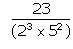(ii) 24/125

(iii) 171/800

(iv) 15/1600

(v) 17/320

(vi) 19/3125

Solution:Clearly either 2 or 5 is not a factor of 23, So given fraction is in its simplest form.

Given fraction already in the form of (2m × 5n).

Hence, the given rational is terminating.

We can written it as:

= 0.115

(ii) 24/125

Denominator = 125 = 53

5 is not a factor of 24, so fraction is in its simplest form.

Also written in the form:

24/125 = 24/(20 x 53 ), which is similar to the form of (2m × 5n).

So, fraction is in the form of (2m × 5n).

Hence, the given rational is terminating.

24/125 = 0.192

(iii) 171/800

Denominator = 180 = 25 x 52

Either 2 or 5 is not a factor of 171, so fraction in its simplest form.

Also written in the form:

24/125 = 171/(25 x 52), which is similar to the form of (2m × 5n).

So, fraction is in the form of (2m × 5n).

Hence, the given rational is terminating.

171/800 = 0.21375

(iv) 15/1600

Denominator = 1600 = 26 x 52

Either 2 or 5 is not a factor of 15.

So the fraction in its simplest form.

Also written in the form:

15/1600 = 15/(26 x 52), which is similar to the form of (2m × 5n).

So, fraction is in the form of (2m × 5n).

Hence, the given rational is terminating.

15/1600 = 0.009375

(v) 17/320

Denominator = 320 = 26 x 5

Either 2 or 5 is not a factor of 17.

So the fraction in its simplest form.

Also written in the form:

17/320= 17/(26 x 5), which is similar to the form of (2m × 5n).

So, fraction is in the form of (2m × 5n).

Hence, the given rational is terminating.

17/320 = 0.053125

(vi) 19/3125

Denominator = 3125 = 55

Either 2 or 5 is not a factor of 19.

So the fraction in its simplest form.

Also written in the form:

19/3125 = 19/(20 x 55), which is similar to the form of (2m × 5n).

So, fraction is in the form of (2m × 5n).

Hence, the given rational is terminating.

19/3125 = 0.00608

Question 2: Without actual division, show that each of the following rational numbers is a non-terminating repeating decimal:

(i)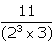(ii)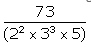(iii)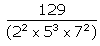(v)77/210
(vi) 32/147
(vii) 29/343
(viii) 64/455

Solution:

(i)2 or 3 is not a factor of 11, so fraction is in its simplest form.

And, (23 x 3) ≠ (2m x 5n)

Hence, the given fraction is non-terminating repeating decimal.

(ii)2, 3 or 5 is not a factor of 73, so fraction is in its simplest form.

And, (22 × 33 × 5) ≠ (2m x 5n)

Hence, the given rational is non-terminating repeating decimal.

(iii)2, 5 or 7 is not a factor of 129, so fraction is in its simplest form.

And, (22 × 57 × 75) ≠ (2m x 5n)

Hence, given fraction is non-terminating repeating decimal.

(iv)9/35

5 or 7 is not a factor of 9, so fraction is in its simplest form.

And, (5 × 7) ≠ (2m x 5n)

Hence, the given fraction is non-terminating repeating decimal.

(v)77/210

2, 3 or 5 is not a factor of 11, so given fraction is in its simplest form.

And, (2 × 3 × 5) ≠ (2m x 5n)

Hence, the given fraction is non-terminating repeating decimal.

(vi) 32/147

3 or 7 is not a factor of 32, so fraction is in its simplest form.

And, (3 × 72) ≠ (2m x 5n)

Hence, the given fraction is non-terminating repeating decimal.

(vii) 29/343

7 is not a factor of 29, so fraction is in its simplest form. And,  73 ≠ (2m x 5n)

Hence, the given fraction is non-terminating repeating decimal.

(viii) 64/455

5, 7 or 13 is not a factor of 64, so it is in its simplest form. And, (5 × 7 × 13) ≠ (2m x 5n)

Hence, the given fraction is non-terminating repeating decimal.

Question 3: Express each of the following as a fraction in simplest form: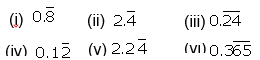Solution:

(i)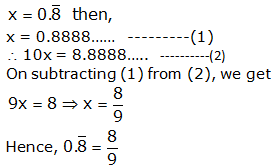(ii)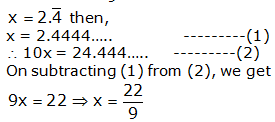(iii)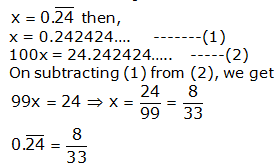(iv)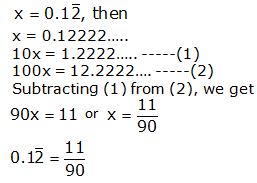(v)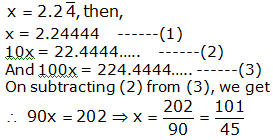(vi)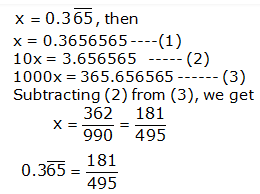## Exercise 1D Page No: 33

Question 1: Define (i) rational numbers, (ii) irrational numbers, (iii) real numbers.

Solution:

Rational numbers: The numbers of the form p/q , where p and q are integers and q ≠ 0.

Irrational numbers: The numbers which when expressed in decimal form and expressible as non-terminating and non-repeating decimals.

Real numbers: Combination of rational and irrational numbers.

Question 2: Classify the following numbers as rational or irrational: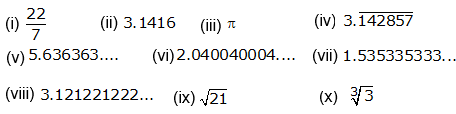Solution:

(i) 22/7 is a rational number.

(ii) 3.1416 is a rational number.

It is a terminating decimal and non-repeating decimal.

(iii) π is an irrational number.

It is a non-terminating and non-repeating decimal.

(iv) $3.\overline{142857}$

A rational number. Non-terminating repeating decimal.

(v) 5.636363…

A rational number. A non-terminating repeating decimal.

(vi) 2.040040004…

An irrational number. It is a non-terminating and non-repeating decimal.

(vii) 1.535335333…

An irrational number. A non-terminating and non-repeating decimal.

(viii) 3.121221222…

An irrational number. A non-terminating and non-repeating decimal.

(ix)$\sqrt{21}$

An irrational number.

21 = 3 × 7 is an irrational number. And 3 and 7 are prime and irrational numbers.

(x)$\sqrt{3}$

An irrational number.

3 is a prime number. So, 3 is an irrational number.

Question 3: Prove that each of the following numbers is irrational.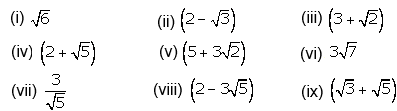Solution:

(i) √6

Let us suppose that √6 is a rational number.

There exists two co-prime numbers, say p and q

So, √6 = p/q

Squaring both sides, we get

6 = p2/q2

or 6q2 = p2 …(1)

Which shows that, p² is divisible by 6

This implies, p is divisible by 6

Let p = 6a for some integer a

Equation (1) implies: 6q² = 36a²

⇨ q² = 6a²

q² is also divisible by 6

⇨ q is divisible by 6

6 is common factors of p and q

But this contradicts the fact that p and q have no common factor

Our assumption is wrong. Thus, √6 is irrational

(ii) (2 – √3)

Let us assume that (2 – √3) is a rational.

Subtract given number form 2, considering 2 is a rational number.

As we know, Difference of two rational numbers is a rational.

So, 2 – (2 – √3 ) is rational

⇨ √3 is rational

Thus, (2 – √3) is an irrational.

(iii) (3 + √2 )

Let us assume that (3 + √2 ) is rational.

Subtract 3 form given number, considering 3 is a rational number.

As we know, Difference of two rational numbers is a rational.

(3 + √2 ) – 3 is rational

⇨ √2 is rational

Which is contradictory to our assumption.

So, (3 + √2 ) is irrational

(iv) (2 + √5 )

Let us assume that (2 + √5 ) is rational.

Subtract 2 form given number, considering 2 is a rational number.

As we know, Difference of two rational numbers is a rational.

(2 + √5) – 2 is rational

⇨ √5 is rational

Which is contradictory to our assumption.

So, (2 + √5) is irrational

(v) (5 + 3√2 )

Let us assume that (5 + 3√2 ) is rational.

Subtract 5 form given number, considering 5 is a rational number.

As we know, Difference of two rational numbers is a rational.

(5 + 3√2 ) – 5 is rational

⇨ 3√2 is rational

And, 3 is rational and √2 is rational.

(Product of two rational numbers is rational)

⇨√2 is rational

Which is contradictory to our assumption.

So, (5 + 3√2 ) is irrational

(vi) 3√7

Let us assume that 3√7 is rational

Here 3 is rational and √7 is rational.

As we know, Product of two rational numbers is rational.

But √7 is rational, which is contradictory to our assumption.

So, 3√7 is irrational

(vii) 3 / √5

Let us assume that 3 / √5 is rational

3 / √5 x √5/√5 = 3√5/5

Where 3√5/5 is rational

Which shows that 3/5 is rational and √5 is rational.

But the fact is √5 is an irrational.

Our assumption is wrong, and

3 / √5 is irrational

(viii)(2 – 3√5)

Let us assume that 2 – 3√5 is rational.

Subtract given number form 2, considering 2 is a rational number.

As we know, Difference of two rational numbers is a rational.

2 – (2 – 3√5) is rational

⇨ 3√5 is rational

Above result is possible if 3 is rational and √5 is rational.

Because, product of two rational numbers is rational

But the fact is √5 is an irrational

Our assumption is wrong, and

(2 – 3√5) is irrational

(ix) (√3 + √5)

Let us assume that √3 + √5 is rational

On squaring, we get

(√3 + √5)² is rational

⇨ 3 + 2√3 x √5 + 5 is rational

⇨ 8 + 2√15 is rational

Subtract 8 from above result, considering 8 is a rational number.

As we know, Difference of two rational numbers is a rational.

⇨ 8 + 2√15 – 8 is rational

⇨ 2√15 is rational

Which is only possible if 2 is rational and √15 is rational.

The fact is √15 is not a rational number.

Our assumption is wrong, and

(√3 + √5) is irrational.

Question 4. Prove that 1/√3 is irrational.

Solution:

Let us assume that 1/√3 is rational

⇨ 1/√3 x √3/ √3 = √3/3 is rational

Which is only possible if 1/3 is rational and √3 is rational. As we know that, Product of two rational numbers is rational

But the fact is, √3 is an irrational.

Which is contradictory to our assumption.

which implies 1/√3 is irrational. Hence proved.

Question 5: (i) Give an example of two irrationals whose sum is rational.

(ii) Give an example of two irrationals whose product is rational.

Solution:

(i) Let us consider two numbers 2 + √3 and 2 – √3 which are irrationals

their sum = (2 + √3) + (2 – √3) = 4; Which is a rational numbers.

(ii) Let us consider two numbers 3 + √2 and 3 – √2 which are irrationals.

their product = (3 + √2) (3 – √2)

= (3)² – (√2)²

= 9 – 2 = 7; which is a rational number.

Question 6.

State whether the given statement is true or false.

(i) The sum of two rationals is always rational.

(ii) The product of two rationals is always rational.

(iii) The sum of two irrationals is always an irrational.

(iv) The product of two irrationals is always an irrational.

(v) The sum of a rational and an irrational is irrational.

(vi) The product of a rational and an irrational is irrational.

Solution:

(i) True.

(ii) True.

(iii) False.

Reason:

Sum of two irrational can be rational. Let us take an example,

Consider two irrational numbers: (3 + √2) and (3 – √2)

Sum: (3 + √2) + (3 – √2) = 3 + √2 + 3 – √2 = 6 which is rational.

(iv) False.

Reason:

Product of two irrational numbers can be rational. Let us take an example,

Consider two irrational numbers: (3 + √2) and (3 – √2)

Product: (3 + √2)(3 – √2 ) = (3)2 – (√2 )2 = 9 – 2 = 7; which is rational

(v) True.

(vi) True.

Question 7. Prove that (2√3 – 1) is an irrational number.

Solution:

Let us assume that (2√3 – 1) is a rational.

Add 1 to the above number, considering 1 is a rational number.

As we know, sum of two rational numbers is a rational.

Sum = 2√3 – 1 + 1 = 2√3

Which is only possible if 2 is rational and √3 is rational.

As we know that product of two rational numbers is rational.

But the fact is √3 is an irrational number which contradicts to our assumption.

So, (2√3 – 1) is an irrational.

Question 8. Prove that (4 – 5√2 ) is an irrational number.

Solution:

Let us assume that (4 – 5√2 ) is a rational.

Subtract given number form 4, considering 4 is a rational number.

As we know, Difference of two rational numbers is a rational.

4 – (4 – 5√2 ) is rational

⇨ 5√2 is rational

Which is only possible if 5 is rational and √2 is rational

As we know, product of two rational number is rational.

But the fact is √2 is an irrational.

Which is contradict to our assumption.

Hence, 4 – 5√2 is irrational. Hence Proved.

Question 9. Prove that (5 – 2√3) is an irrational number.

Solution:

Let us assume that (5 – 2√3) is a rational.

Subtract given number form 5, considering 5 is a rational number.

As we know, Difference of two rational numbers is a rational.

⇨ 5 – (5 – 2√3) is rational

⇨ 2√3 is rational

Which is only possible if 2 is rational and √3 is rational

As we know, product of two rational number is rational.

But the fact is √3 is an irrational.

Which is contradict to our assumption.

(5 – 2√3) is an irrational number.

Question 10: Prove that 5√2 is irrational.

Solution:

Let us assume that 5√2 is a rational.

Which is only possible if 5 is rational and √2 is rational

As we know, product of two rational number is rational.

But the fact is √2 is an irrational.

Which is contradict to our assumption.

5√2 is an irrational. Hence proved.

Question 11: Prove that 2/√7 is irrational.

Solution:

Let us assume that 2/√7 is a rational number.

2/√7 x √7/√7 = 2√7/7 is a rational number

Which is only possible if 2/7 is rational and √7 is rational.

But the fact is √7 is an irrational.

Which is contradicts to our assumption.

2/√7 is an irrational number. Hence proved.

## Exercise 1E Page No: 34

Question 1: State Euclid’s division lemma.

Solution:

As per Euclid’s division lemma: For any two positive integers, say a and b, there exit unique integers q and r, such that a = bq + r; where 0 ≤ r < b.

Also written as: Dividend = (Divisor x Quotient) + Remainder

Question 2: State fundamental theorem of Arithmetic.

Solution:

Every composite number can be uniquely expressed as a product of two primes, except for the order in which these prime factors occurs.

Question 3. Express 360 as product of its prime factors.

Solution: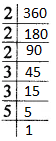360 = 2 x 2 x 2 x 3 x 3 x 5 = 23 x 32 x 5

Question 4: If a and b are two prime numbers, then find HCF (a, b).

Solution:

HCF of two primes is always 1.

HCF (a, b) = 1

Question 5: If a and b are two prime numbers then find LCM (a, b).

Solution:

LCM of two prime numbers ia always the product of these two numbers.

If a and b are two prime numbers, then

LCM (a, b) = ab

Question 6: If the product of two numbers is 1050 and their HCF is 25, find their LCM.

Solution:

Product of two numbers = HCF x LCM …(1)

Given: Product of two numbers = 1050

and HCF = 25

From equation (1), we have

1050 = 25 x LCM

or

LCM = 1050 / 25 = 42

Question 7: What is a composite number?

Solution:

A composite number has more than two factors.

Another definition: A composite number is a number which is not a prime.

Question 8: If a and b are relatively prime then what is their HCF?

Solution:

If a and b are two primes, then their HCF will be 1.

HCF (a, b) = 1

Question 9.

If the rational number a/b has a terminating b decimal expansion, what is the condition to be satisfied by b.

Solution:

Since a/b is a rational number and it has terminating decimal “b” will in the form 2m x 5n where m and n are some non-negative integers.

Question 10: Simplify

$\frac{2\sqrt{45} + 3\sqrt{20}}{2\sqrt{5}}$

Solution:

$\frac{2\sqrt{45} + 3\sqrt{20}}{2\sqrt{5}}$ = $\frac{2\sqrt{9\times5} + 3\sqrt{4\times5}}{2\sqrt{5}}$ $\frac{2\times3\sqrt{5} + 3\times2\sqrt{5}}{2\sqrt{5}}$ $\frac{6\sqrt{5} + 6\sqrt{5}}{2\sqrt{5}}$

= 6

Question 11: Write the decimal expansion of

$\frac{73}{2^{4}\times5^{3}}$

Solution:

$\frac{73}{2^{4}\times5^{3}}$ = $\frac{73}{16\times125}$

= 73/1000

= 0.073

Question 12: Show that there is no value of n for which (2n x 5n) ends in 5.

Solution:

2n x 5n can also be written as

2n x 5n = (2 x 5)n = (10)n

Which always ends in a zero

There is no value of n for which (2n x 5n) ends in 5

Question 13: Is it possible to have two numbers whose HCF is 25 and LCM is 520?

Solution:

HCF is always a factor is its LCM.

Given: HCF is 25 and LCM is 520

But 25 is not a factor of 520

It is not possible to have two numbers having HCF is 25 and LCM is 520.

Question 14: Give an example of two irrationals whose sum is rational.

Solution:

Let us consider two irrational number be (5 + √3) and (5 – √3).

Sum = (5 + √3) + (5 – √3) = 10

Which is a rational number.

Question 15: Give an example of two irrationals whose product is rational.

Solution:

Let us consider that the two irrational number be (3 + √2) and (3 – √2)

Product = (3 + √2) (3 – √2)

= (3)² – (√2)² = 7

Which is a rational number.

Question 16: If a and b are relatively prime, what is their LCM?

Solution: Two relatively prime numbers do not have a common factor other than 1.

i.e, HCF = 1.

We know HCF × LCM = product of numbers.

LCM = Product of numbers

If a and b are two numbers then

LCM = a × b = ab.

Question 17: The LCM of two numbers is 1200. Show that the HCF of these numbers cannot be 500. Why?

Solution:

Given: LCM of two numbers = 1200

HCF should divide LCM exactly.

Using Euclid’s division lemma – a = bq + r. where q is the quotient, r is the remainder and b is the divisor.

Let us say, a = 1200 and b = 500.

If HCF divides LCM completely, then remainder is zero.

Here 1200 = 500(2) + 200

r = 200 ≠ 0

Question 18: Express $0.\overline{4}$ as a rational number in simplest form.

Solution: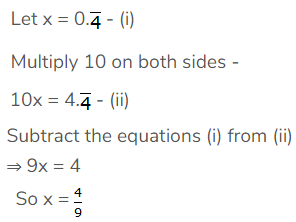19. Express $0.\overline{23}$ as a rational number in simplest form.

Solution: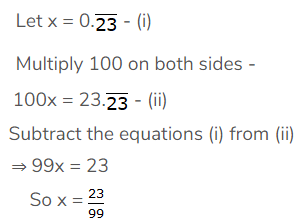Question 20: Express why 0.15015001500015… is an irrational number.

Solution:

As we know, Irrational numbers are non-terminating non-recurring decimals.

Thus, 0.15015001500015 … is an irrational number.

Question 21: Show that √2/3 is irrational.

Solution: Let us assume that √2/3 is rational

Which is possible if 1/3 is rational and √2 is rational

But the fact is √2 is an irrational.

Which is contradict, our assumption is wrong.

√2/3 is irrational.

Question 22: Write a rational number between √3 and 2.

Solution:

The value of √3 is 1.73.

A rational number between √3 and 2 is 1.8.

Question 23: Explain why $3.\overline{1416}$is a rational number.

Solution:

$3.\overline{1416}$ is a non – terminating repeating decimal.

So it is a rational number.

## RS Aggarwal Solutions for Class 10 Maths Chapter 1 Real Numbers

In this chapter students will deal with some of the important concepts of real numbers are listed below:

• Euclid’s Division Lemma
• Some Applications of Euclid’s Division Lemma
• Fundamental Theorem of Arithmetic
• An Important property: Product of two given numbers = Product of theri HCF and LCM
• Rational Numbers
• Irrational Numbers
• Important results on Irrational

### Key Features of RS Aggarwal Solutions for Class 10 Maths Chapter 1 Real Numbers

1. Based on the latest CBSE syllabus
2. Free study materials.
3. Easy for quick revision
4. Help to clear doubts and score more marks in the exams.
5. These solutions will help the students to strengthen their foundation on real numbers.